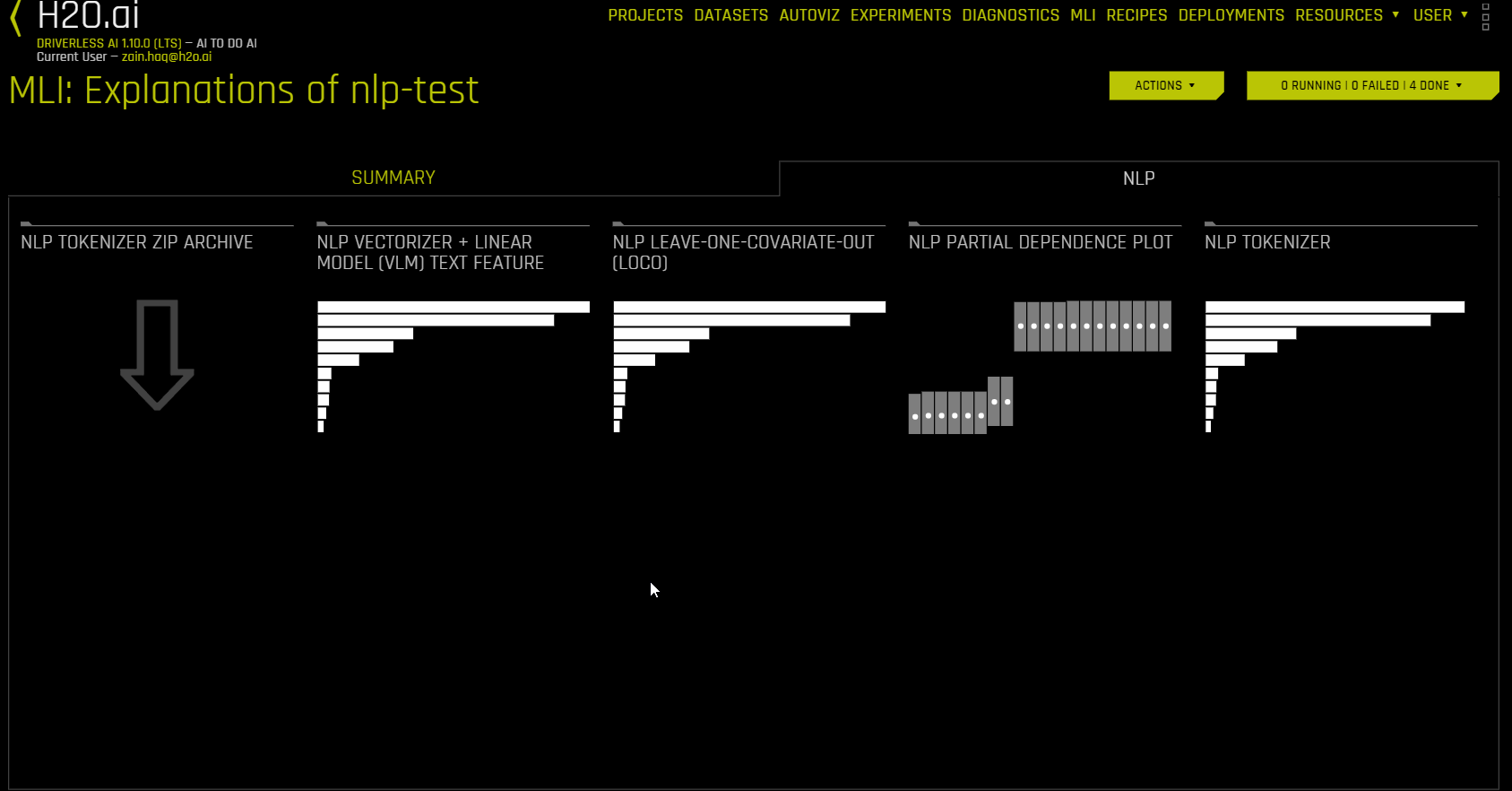# Understanding the Model Interpretation Page¶

This page describes the various interpretations available from the Machine Learning Interpretability (MLI) explanations page for non-time-series experiments.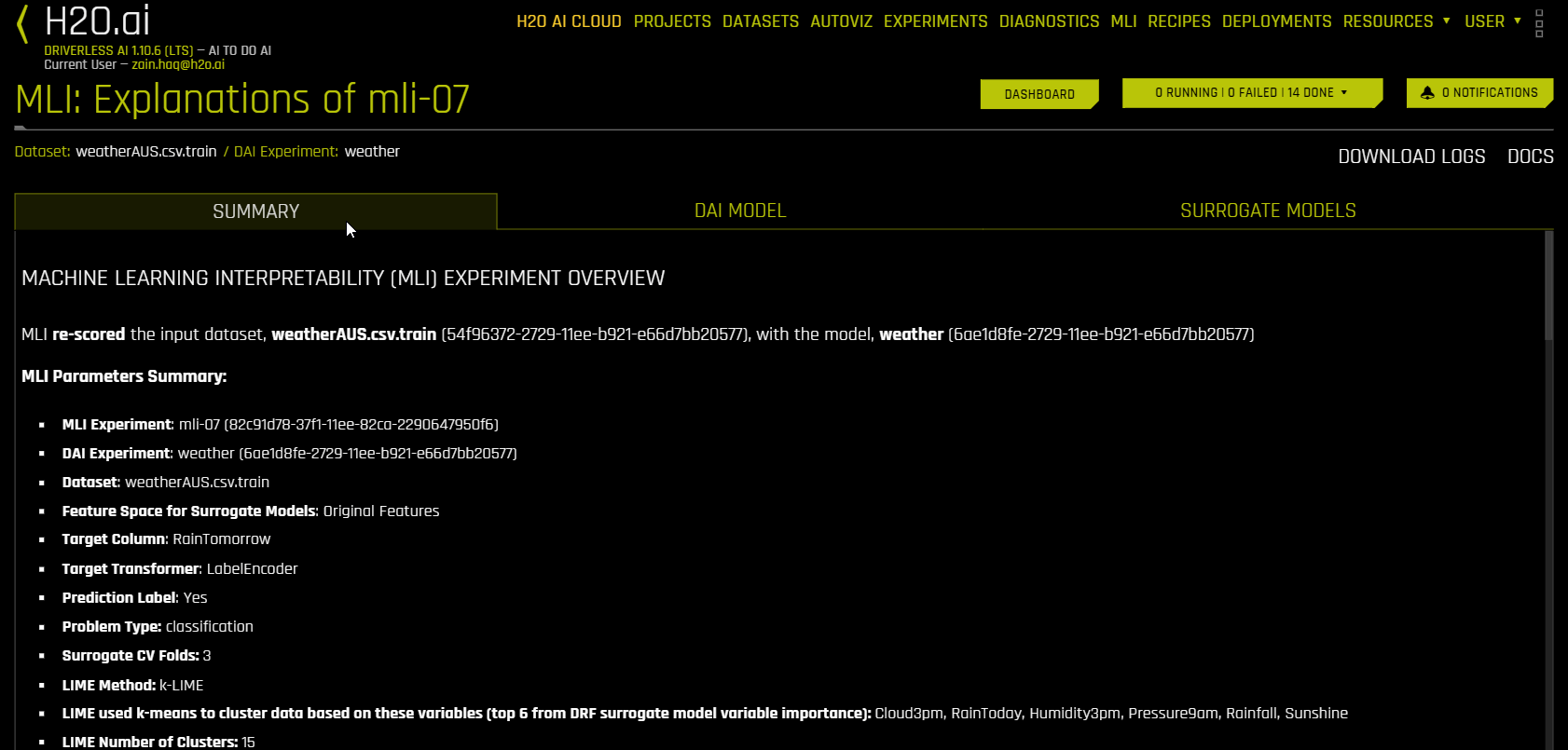The explanations page is organized into four tabs:

You can click the Dashboard button to view a dashboard with an overview of the interpretations built using Driverless AI and surrogate models. For more information, see MLI Dashboard.

The task bar lists the status and logs of MLI explainers.

From the explanations page, you can view the official DAI MLI documentation by clicking Docs and download MLI logs by clicking Download Logs.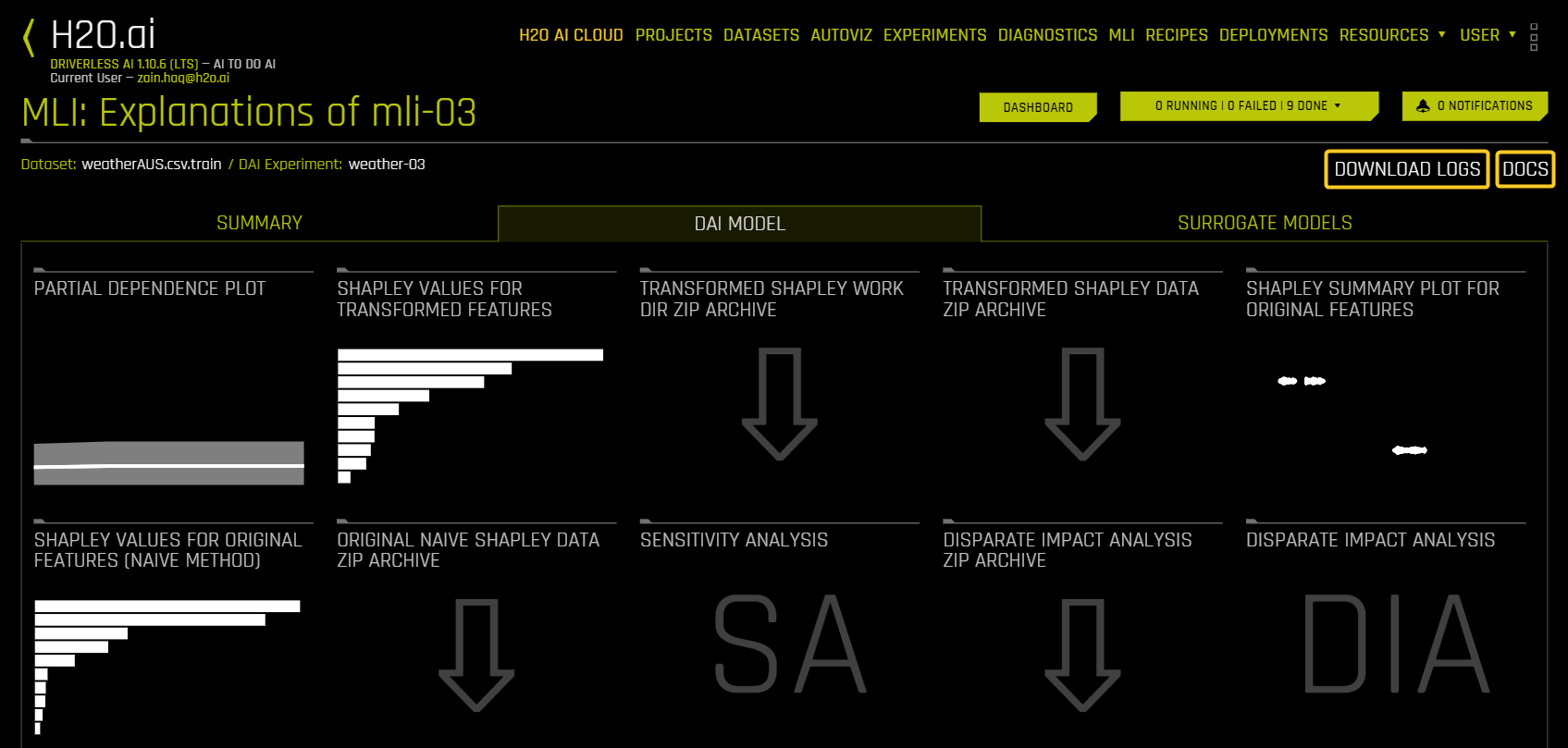Explainer detail page actions

The following is a list of common actions that are available when viewing an explainer detail page. Note that some of these actions may not be available for certain explainers.

• Video tutorial: You can access video tutorials through the UI for the following explainers by clicking the play arrow icon.

• Partial Dependence (PDP)

• Shapley for transformed features

• Shapley for original features

• Shapley summary plot

• Disparate Impact Analysis (DIA)

• Sensitivity Analysis

• Surrogate decision tree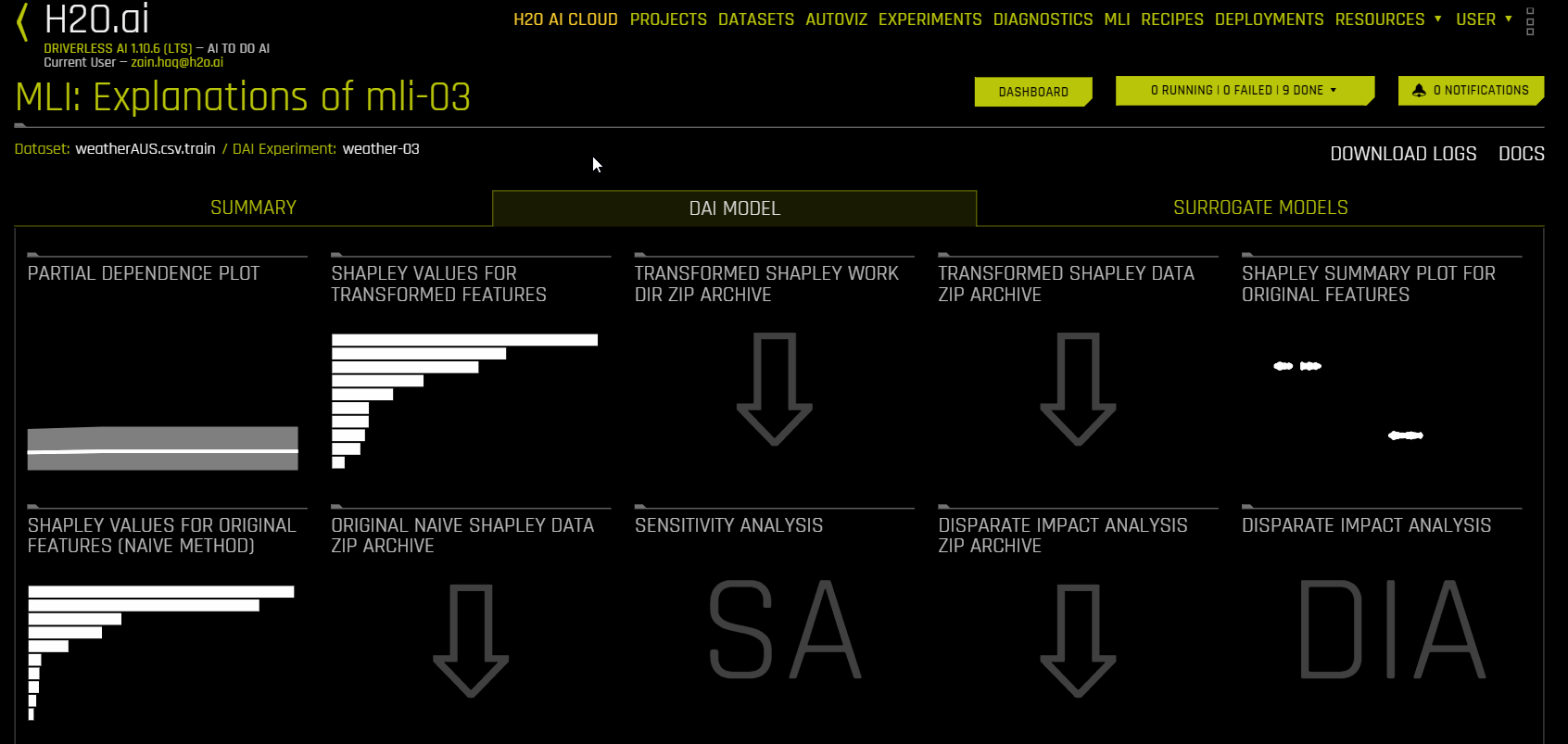• Help: View a description of the explainer.

• Close: Exit to the interpretation page.

You can view a list of recent notifications from the interpretation page and explainer detail pages by clicking the Notifications button. Notifications remain present in the notification center unless they are manually cleared. The notification center displays general notifications as well as notifications raised by specific explainers.

## Summary Tab¶

The Summary tab provides an overview of the interpretation, including the dataset and Driverless AI experiment name (if available) that were used for the interpretation along with the feature space (original or transformed), target column, problem type, and k-Lime information. If the interpretation was created from a Driverless AI model, then a table with the Driverless AI model summary is also included along with the top variables for the model.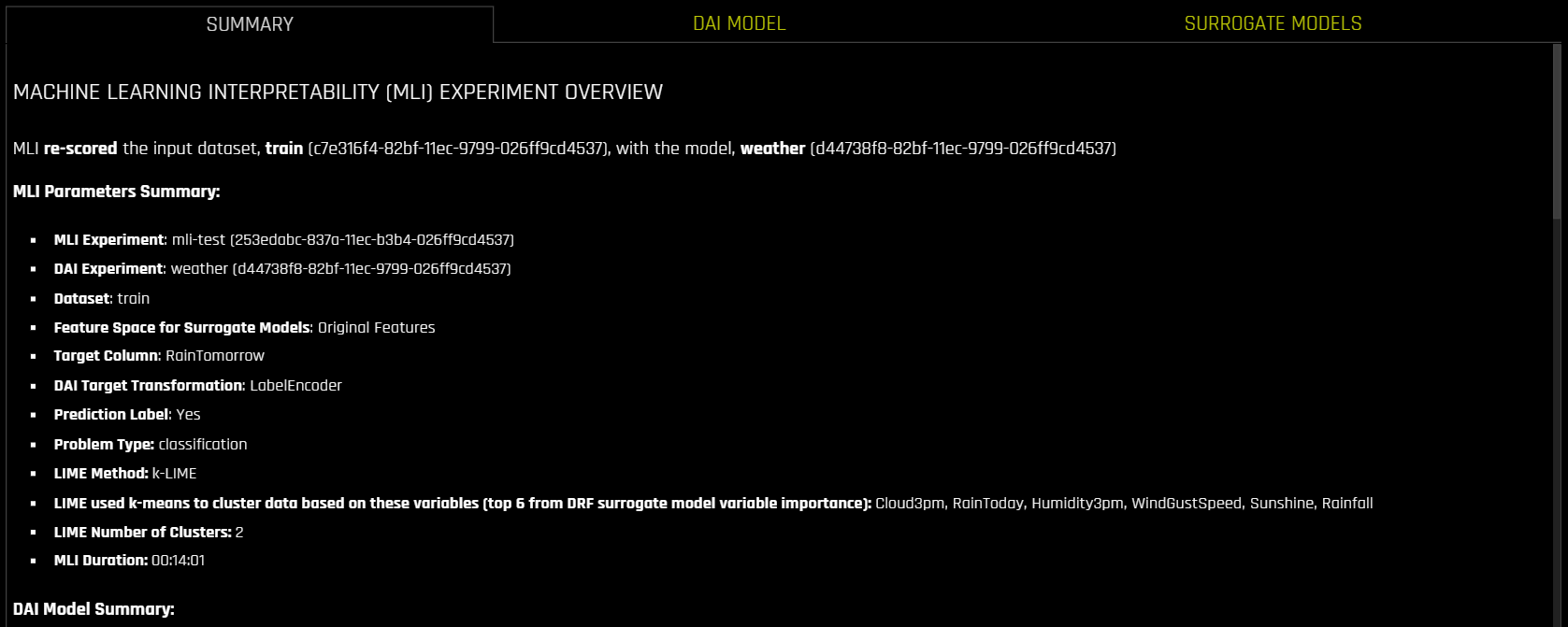## Interpretations Using Driverless AI Model (DAI Model Tab)¶

The DAI Model tab is organized into tiles for each interpretation method. To view a specific plot, click the tile for the plot that you want to view.

For binary classification and regression experiments, this tab includes Feature Importance and Shapley (not supported for RuleFit and TensorFlow models) plots for original and transformed features as well as Partial Dependence/ICE, Disparate Impact Analysis (DIA), Sensitivity Analysis, NLP Tokens and NLP LOCO (for text experiments), and Permutation Feature Importance (if the autodoc_include_permutation_feature_importance configuration option is enabled) plots. For multiclass classification experiments, this tab includes Feature Importance and Shapley plots for original and transformed features.

The following is a list of the interpretation plots available from the Driverless AI Model tab: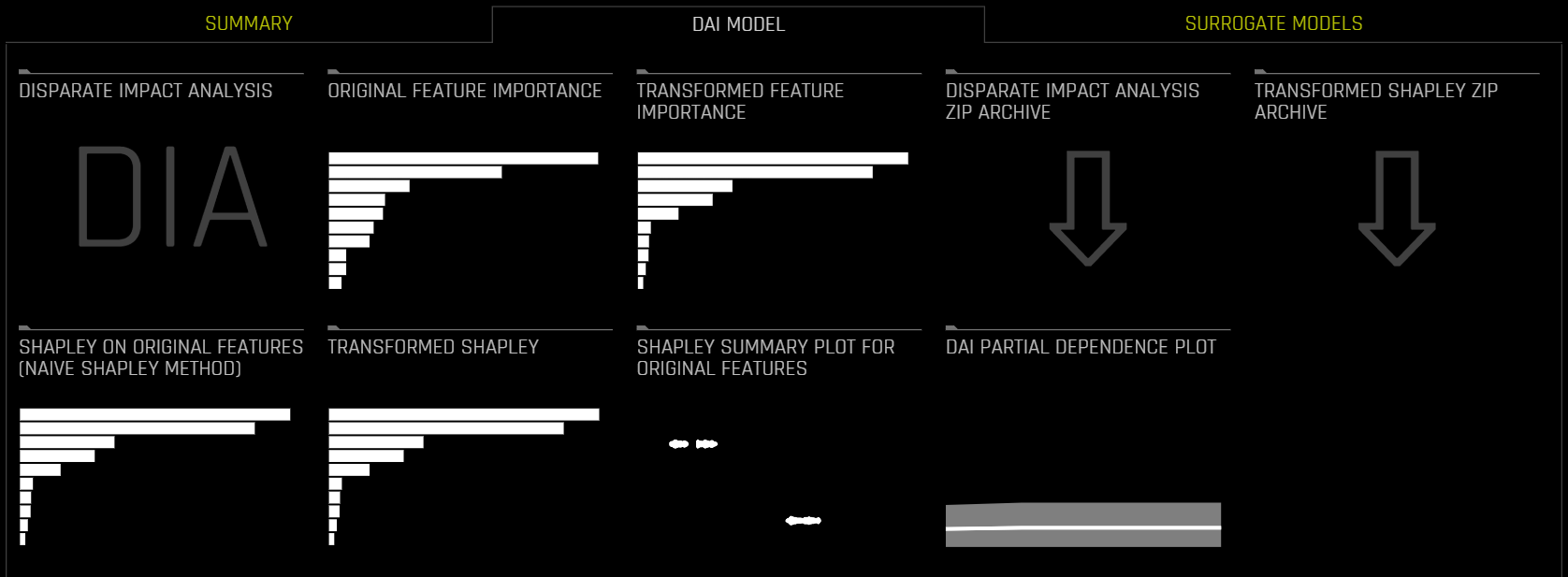Notes:

• When running DIA on an external model, the DAI Model tab on the the interpretation page is renamed to 3rd Party Model.

• Shapley plots are not supported for RuleFit, FTRL, and TensorFlow models.

• By default, the Shapley on original features is calculated using the Naive Shapley method (see notes). To enable the calculations using Kernel Explainer method, enable Original Kernel SHAP explainer in recipes.

• Shapley plots are only supported for those BYOR (custom) models that implement the has_pred_contribs method (and return True) and implement proper handling of the argument pred_contribs=True in the predict method.

• The Permutation-based feature importance plot is only available when the autodoc_include_permutation_feature_importance configuration option is enabled when starting Driverless AI or when starting the MLI experiment (enable AutoDoc from the recipe tab and include_permutation_feature_importance from MLI AutoDoc expert settings when launching the MLI job).

• On the Feature Importance and Shapley plots, the transformed feature names are encoded as follows:

<transformation/gene_details_id>_<transformation_name>:<orig>:<…>:<orig>.<extra>

So in 32_NumToCatTE:BILL_AMT1:EDUCATION:MARRIAGE:SEX.0, for example:

• 32_ is the transformation index for specific transformation parameters.

• NumToCatTE is the transformation type.

• BILL_AMT1:EDUCATION:MARRIAGE:SEX represent original features used.

• 0 represents the likelihood encoding for target after grouping by features (shown here as BILL_AMT1, EDUCATION, MARRIAGE and SEX) and making out-of-fold estimates. For multiclass experiments, this value is > 0. For binary experiments, this value is always 0.

## Interpretations Using Surrogate Model (Surrogate Model Tab)¶

A surrogate model is a data mining and engineering technique in which a generally simpler model is used to explain another, usually more complex, model or phenomenon. For example, the decision tree surrogate model is trained to predict the predictions of the more complex Driverless AI model using the original model inputs. The trained surrogate model enables a heuristic understanding (i.e., not a mathematically precise understanding) of the mechanisms of the highly complex and nonlinear Driverless AI model.

The Surrogate Model tab is organized into tiles for each interpretation method. To view a specific plot, click the tile for the plot that you want to view. For binary classification and regression experiments, this tab includes K-LIME/LIME-SUP and Decision Tree plots as well as Feature Importance, Partial Dependence, and LOCO plots for the Random Forest surrogate model. For more information on these plots, see Surrogate Model Plots.

The following is a list of the interpretation plots from Surrogate Models: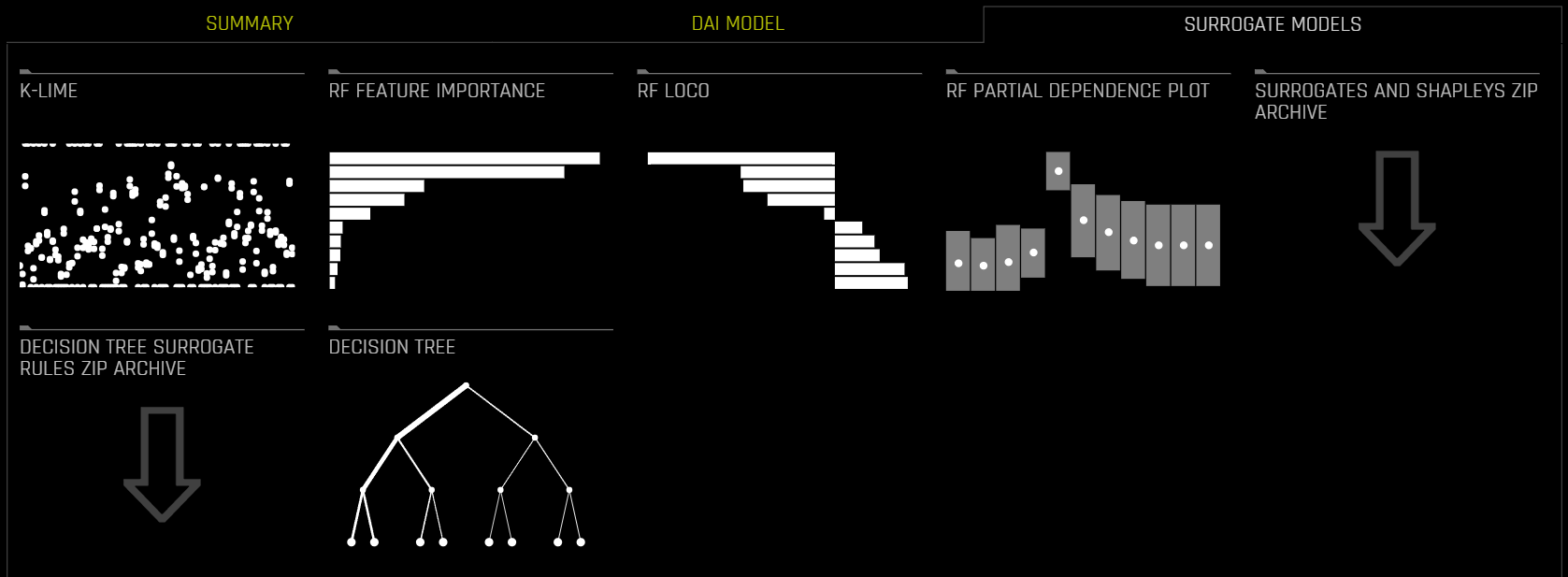Note: For multiclass classification experiments, only the Decision Tree and Random Forest Feature Importance plots are available in this tab.

## Interpretations Using NLP Dataset (NLP Tab)¶

The NLP tab is only visible for natural language processing (NLP) problems and is organized into tiles for each interpretation method. To view a specific plot, click the tile for the plot that you want to view

The following is a list of the interpretation plots available from the NLP tab: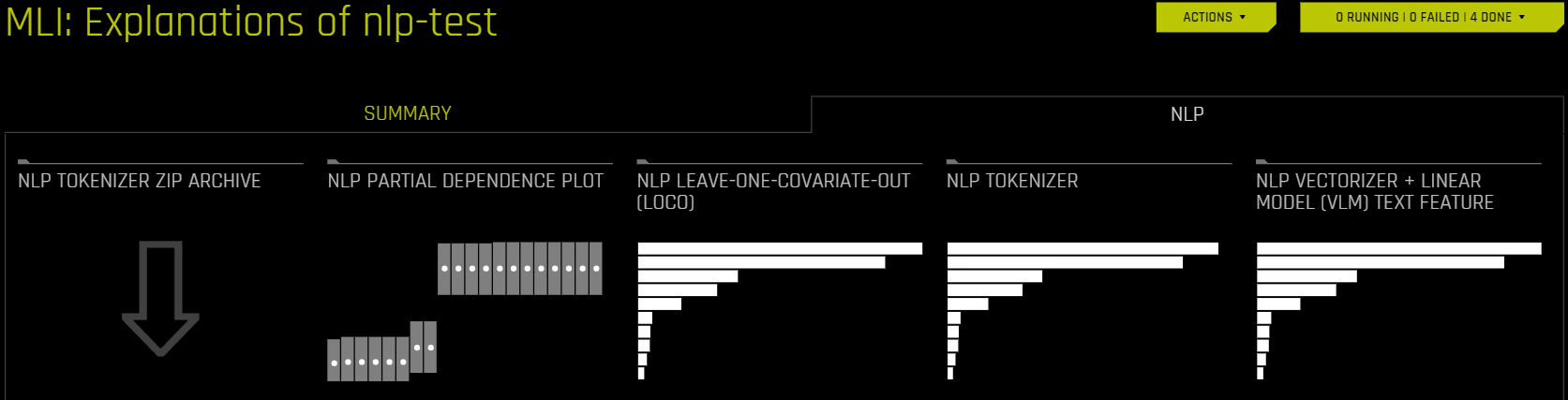## MLI Dashboard¶

To view a dashboard with an overview of the interpretations built using Driverless AI and surrogate models, click the Dashboard button.

The MLI dashboard is organized into two tabs for interpretations built using Driverless AI models and interpretations built using surrogate models:

• The DAI Model tab of the MLI Dashboard provides an overview of the following MLI explainers:

• Shapley Values for Transformed Features

• Shapley Values for Original Features (Naive Method)

• Shapley Summary Plot for Original Features (Naive Shapley Method)

• Partial Dependence Plot

• The Surrogate Models tab of the MLI Dashboard provides an overview of the following MLI explainers:

• K-LIME/LIME-SUP

• Surrogate Random Forest Feature Importance

• Surrogate Decision Tree

• Surrogate Random Forest Partial Dependence Plot

Note: Depending on the experiment type, certain MLI explainers may not be visible in the MLI Dashboard.

### Dashboard usage guide¶

The following subsections describe how to use the MLI Dashboard.

#### Search by row number¶

To search by a specific row number, enter the row number and click the Search button. Searching by a specific row number can provide insight into specific predictions when comparing the different MLI explainer plots. You can also view the specified row in detail by clicking the Show Row button.

#### View explanations¶

You can view explanations from either tab by clicking the Explanations button located in the upper-right corner. For more information, see Viewing Explanations.

#### View MLI explainer in detail¶

To view an individual MLI explainer on the MLI Dashboard in detail, click the arrow icon to the left of the explainer name:

You can download an image in PNG format of the current MLI explainer view by clicking the arrow to the right of the explainer name. Note that if you modify the view by searching by a specific row, the downloaded image reflects the modified view.

The MLI explainers tasks and logs for non-time-series experiments can be viewed from the status manager: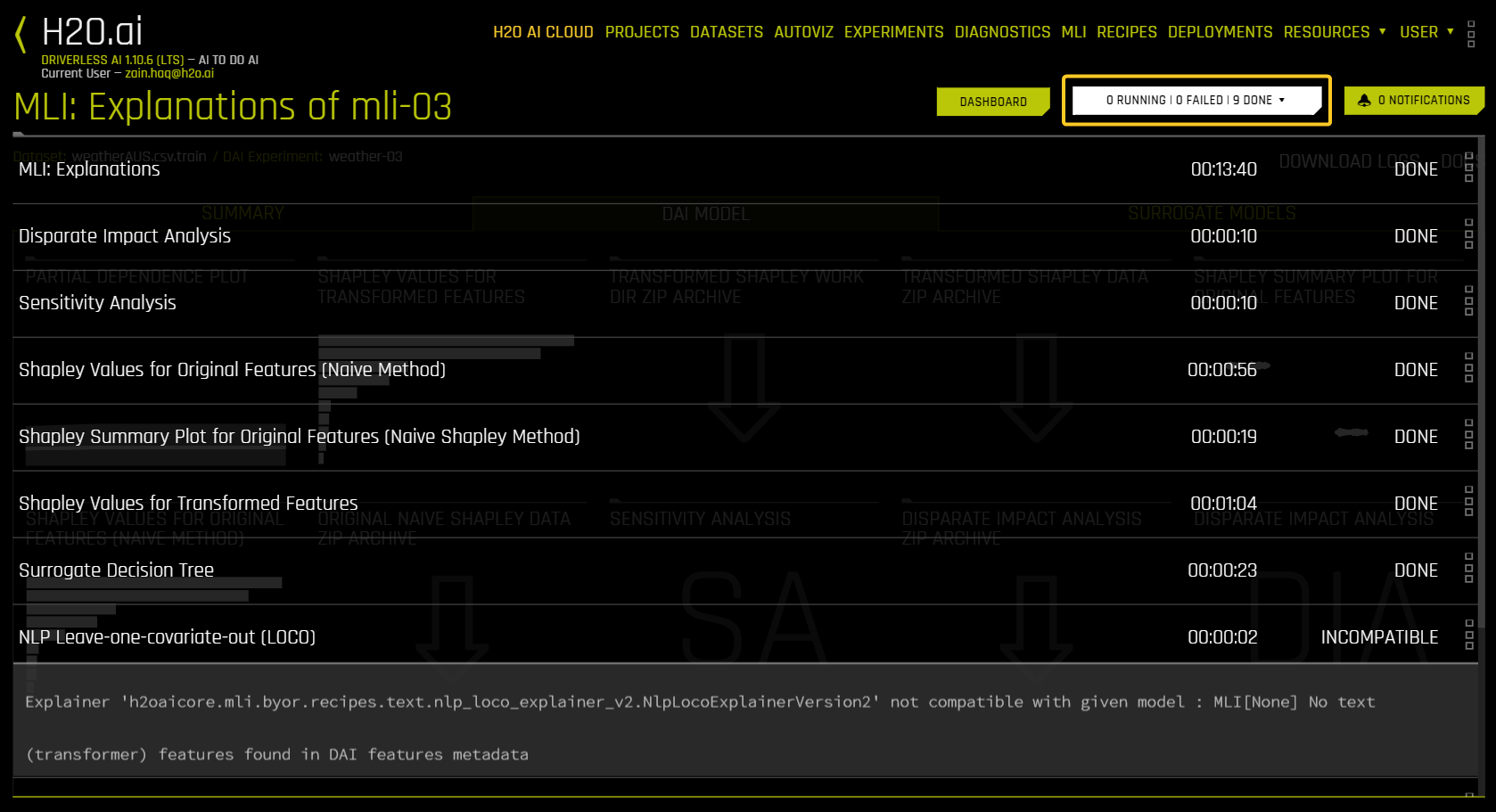## DAI Model Plots¶

This section describes the plots that are available in the DAI Model Tab.

### Feature Importance (Original and Transformed Features)¶

This plot is available for all models for binary classification, multiclass classification, and regression experiments.

This plot shows the Driverless AI feature importance. Driverless AI feature importance is a measure of the contribution of an input variable to the overall predictions of the Driverless AI model.

### Shapley (Original and Transformed Features)¶

This plot is not available for RuleFit or TensorFlow models. For all other models, this plot is available for binary classification, multiclass classification, and regression experiments.

Shapley explanations are a technique with credible theoretical support that presents consistent global and local variable contributions. Local Shapley values are calculated by tracing single rows of data through a trained tree ensemble and aggregating the contribution of each input variable as the row of data moves through the trained ensemble. Global Shapley values are the average of the absolute Shapley values over every row of a dataset.

Notes: The following notes describe cases in which the sum of the Shapley values does not match the prediction.

• If fast approximation is set to True (the default setting), the Shapley calculation is sped up by approximating predictions. To turn off this setting, navigate to the settings for each Shapley explainer and select DISABLED under the text Speed up predictions with fast predictions and contributions approximations.

• Using the identity_noclip target transformer is recommended if interpretability is important. Otherwise, the inverse of the target transformation needs to be applied to the sum of the Shapley values to obtain the model prediction.

#### Regression versus classification¶

For regression tasks, Shapley values sum to the prediction of the Driverless AI model if the target transformer used is identity_noclip. Otherwise, the sum will be in the target transformer space.

For classification problems, the Shapley values should be summed to the prediction of the Driverless AI model after applying the appropriate link function. In the case of binary classification, the sum of Shapley values (Bias + Contributions) can be passed through a sigmoid function (1 / (1 + exp(-x))) to obtain the prediction. For multiclass classification, the sum of Shapley values (Bias + Contributions) for each class can be passed through a softmax function: exp(z_i) / sum(exp(z_j)) for i = 1, 2, …, n.

#### Methods for calculating Shapley values for transformed and original features¶

Shapley values for transformed features are calculated by tracing individual rows of data through a trained tree ensemble and then aggregating the contribution of each input variable as the row of data moves through the trained ensemble. More information about Shapley for tree-based models is available at https://arxiv.org/abs/1706.06060. Driverless AI directly calls XGBoost and LightGBM SHAP functions to get contributions for transformed features.

Shapley values for original features are approximated from the accompanying Shapley values for transformed features with the Naive Shapley (even split) method. This method makes the assumption that input features to a transformer are independent and splits the contribution evenly among the contributing features. For example, if the transformed feature CVTE:age:income.0 has a Shapley value of 5, then the Shapley value of the original features age and income will be 2.5 each. For ensembles, Shapley values (in the link space) are blended as per the model weights in the ensemble.

Driverless AI MOJO for productionization supports Naive Shapley (even split) approach for original features.

Shapley values for original features can also be calculated with the Kernel Explainer method, which uses a special weighted linear regression to compute the importance of each feature. This can be enabled by using the recipe Original Kernel SHAP explainer. More information about Kernel SHAP is available at http://papers.nips.cc/paper/7062-a-unified-approach-to-interpreting-model-predictions.pdf.

The Showing $$n$$ Features drop-down for Feature Importance and Shapley plots lets you select between original and transformed features. If there are a significant amount of features, they will be organized in numbered pages that can be viewed individually. Note: The provided original values are approximations derived from the accompanying transformed values. For example, if the transformed feature $$feature1\_feature2$$ has a value of 0.5, then the value of the original features ($$feature1$$ and $$feature2$$) will be 0.25.

#### Key terms¶

This section describes several key terms that are used in the Shapley plots.

• Global bias: The average model output over the training dataset. Note that for regression models, this is done in the target transformation space.

• Bias + Contributions: The sum of all signed Shapley contributions, which are offset by the bias term.

### Shapley Summary Plot (Original Features)¶

The Shapley Summary Plot shows original features versus their local Shapley values on a sample of the dataset. Feature values are binned by Shapley values, and the average normalized feature value for each bin is plotted. To see the Shapley value, number of rows, and average normalized feature value for a particular feature bin, hold the pointer over the bin. The legend corresponds to numeric features and maps to their normalized value. Yellow is the lowest value, and deep orange is the highest. You can click on numeric features to see a scatter plot of the actual feature values versus their corresponding Shapley values. Categorical features are shown in grey and do not provide an actual-value scatter plot.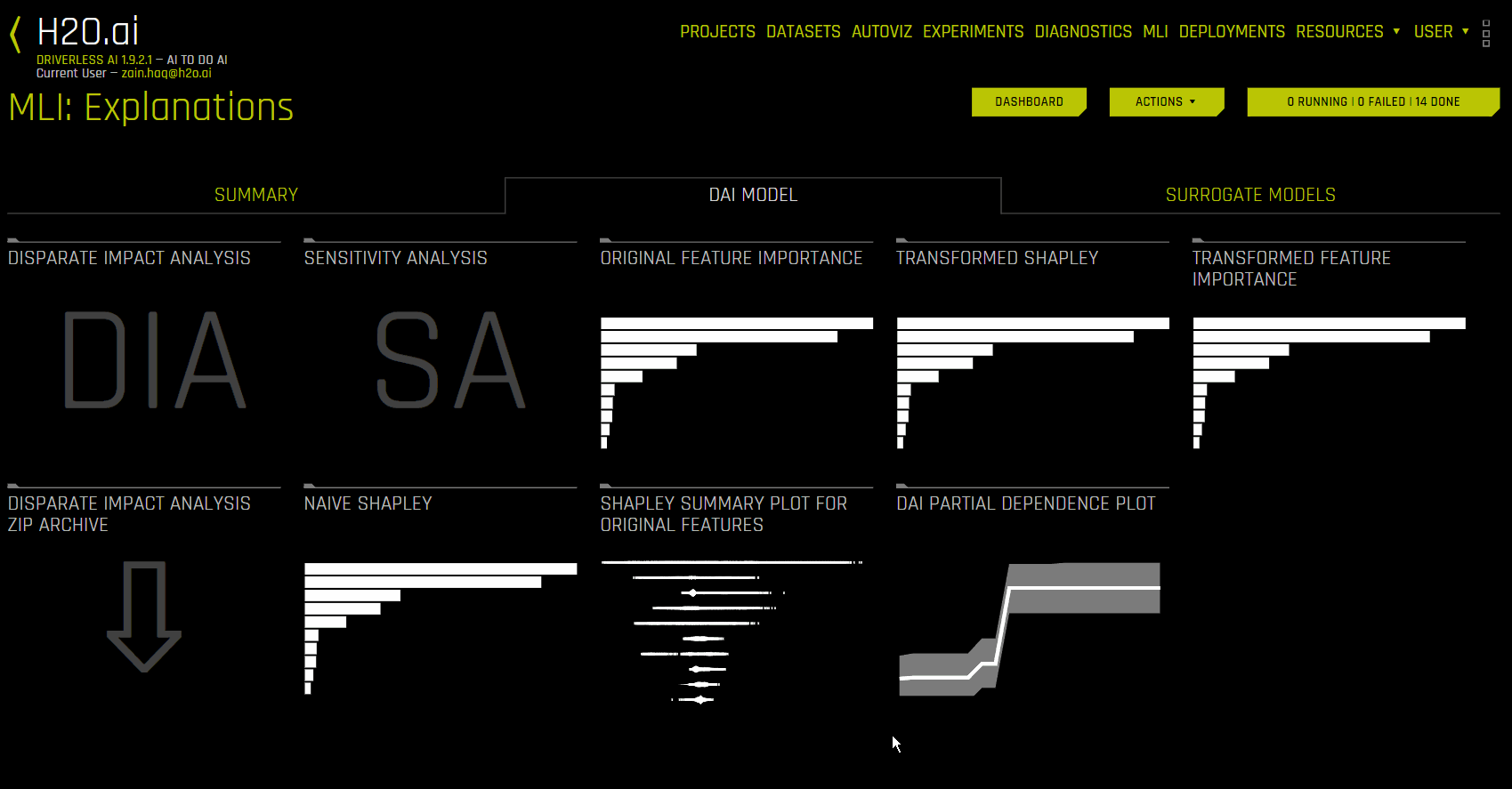Notes:

• The Shapley Summary Plot only shows original features that are used in the Driverless AI model.

• The dataset sample size and the number of bins can be updated in the Interpretation Expert Settings.

• For a list of Shapley Summary Plot explainer expert settings, see Shapley Summary Plot Explainer Settings.

### Partial Dependence (PDP) and Individual Conditional Expectation (ICE)¶

A Partial Dependence and ICE plot is available for both Driverless AI and surrogate models.

#### The Partial Dependence Technique¶

Partial dependence is a measure of the average model prediction with respect to an input variable. Partial dependence plots display how machine-learned response functions change based on the values of an input variable of interest while taking nonlinearity into consideration and averaging out the effects of all other input variables. Partial dependence plots are described in the Elements of Statistical Learning (Hastie et al, 2001). Partial dependence plots enable increased transparency in Driverless AI models and the ability to validate and debug Driverless AI models by comparing a variable’s average predictions across its domain to known standards, domain knowledge, and reasonable expectations.

#### The ICE Technique¶

This plot is available for binary classification and regression models.

A newer adaptation of partial dependence plots called Individual conditional expectation (ICE) plots can be used to create more localized explanations for a single individual by using the same basic ideas as partial dependence plots. ICE Plots were described by Goldstein et al (2015). ICE values are disaggregated partial dependence, but ICE is also a type of nonlinear sensitivity analysis in which the model predictions for a single row are measured while a variable of interest is varied over its domain. ICE plots enable a user to determine whether the model’s treatment of an individual row of data is outside one standard deviation from the average model behavior, whether the treatment of a specific row is valid in comparison to average model behavior, known standards, domain knowledge, and reasonable expectations, and how a model will behave in hypothetical situations where one variable in a selected row is varied across its domain.

Given the row of input data with its corresponding Driverless AI and K-LIME predictions:

debt_to_income_ ratio

credit_ score

savings_acct_ balance

observed_ default

H2OAI_predicted_ default

K-LIME_predicted_ default

30

600

1000

1

0.85

0.9

Taking the Driverless AI model as F(X), assuming credit scores vary from 500 to 800 in the training data, and that increments of 30 are used to plot the ICE curve, ICE is calculated as follows:

$$\text{ICE}_{credit\_score, 500} = F(30, 500, 1000)$$

$$\text{ICE}_{credit\_score, 530} = F(30, 530, 1000)$$

$$\text{ICE}_{credit\_score, 560} = F(30, 560, 1000)$$

$$...$$

$$\text{ICE}_{credit\_score, 800} = F(30, 800, 1000)$$

The one-dimensional partial dependence plots displayed here do not take interactions into account. Large differences in partial dependence and ICE are an indication that strong variable interactions may be present. In this case partial dependence plots may be misleading because average model behavior may not accurately reflect local behavior.

#### Partial Dependence Plot (PDP)¶

This plot is available for binary classification and regression models.

Overlaying ICE plots onto partial dependence plots allow the comparison of the Driverless AI model’s treatment of certain examples or individuals to the model’s average predictions over the domain of an input variable of interest.

This plot shows the partial dependence when a variable is selected and the ICE values when a specific row is selected. Users may select a point on the graph to see the specific value at that point. You can also focus the PDP plot on a specific subset of data by using the slider in the middle of the screen. Partial dependence (yellow) portrays the average prediction behavior of the Driverless AI model across the domain of an input variable along with +/- 1 standard deviation bands. ICE (grey) displays the prediction behavior for an individual row of data when an input variable is toggled across its domain. Currently, partial dependence and ICE plots are only available for the top ten most important original input variables. Categorical variables with 20 or more unique values are never included in these plots.

Notes:

• To use dynamic switching between PDP numeric and categorical binning and UI chart selection in cases where features were used both as numeric and categorical by the experiment, enable the mli_pd_numcat_num_chart config.toml setting. (This setting is enabled by default.) When this setting is enabled, you can specify the threshold for PDP binning and chart selection with the mli_pd_numcat_threshold setting, which defaults to 11.

• The number of out of range / unseen PD or ICE bins can be specified through the PDP explainer oor_grid_resolution expert setting: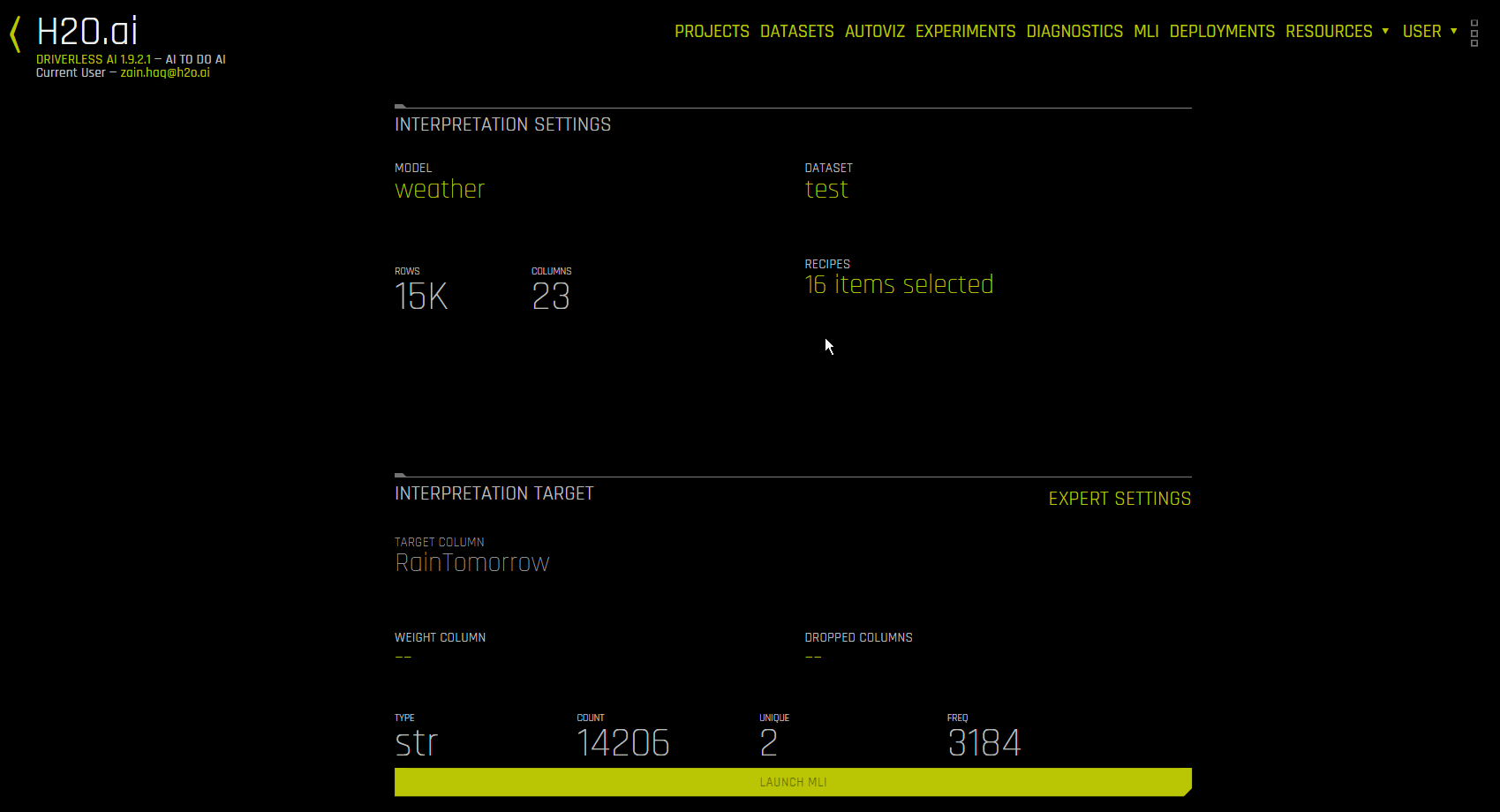##### Partial Dependence On-Demand¶

The PD on-demand option lets you choose an existing or new feature for which to compute Driverless AI PD/ICE from an existing interpretation. With this method, PD/ICE is calculated by an ad hoc explainer, then run and merged to the original DAI PD/ICE representation.

To use the PD on-demand option, click the interpretation you want to use, then click DAI Partial Dependence Plot from the DAI Model tab. On the PD plot page, click the Add Feature button and select the feature(s) you want to calculate PD for. Click Done to confirm your selection. A notification appears at the bottom of the screen once Driverless AI has finished the on-demand computation. To view the computed PD values for a particular feature, click Feature on the PD plot page, then select the feature you want to view PD values for.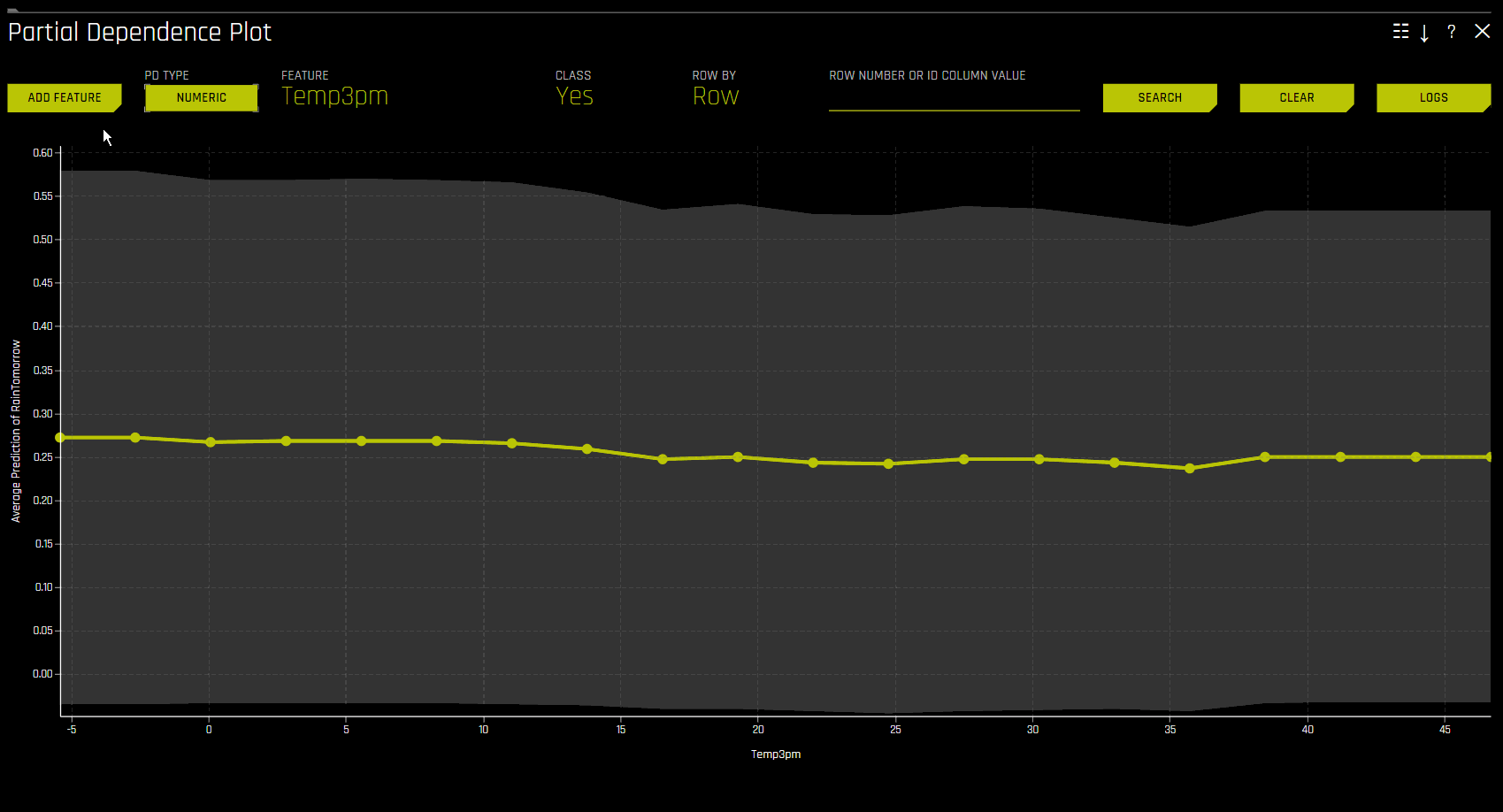### Disparate Impact Analysis (DIA)¶

This plot is available for binary classification and regression models.

DIA is a technique that is used to evaluate fairness. Bias can be introduced to models during the process of collecting, processing, and labeling data—as a result, it is important to determine whether a model is harming certain users by making a significant number of biased decisions.

DIA typically works by comparing aggregate measurements of unprivileged groups to a privileged group. For instance, the proportion of the unprivileged group that receives the potentially harmful outcome is divided by the proportion of the privileged group that receives the same outcome—the resulting proportion is then used to determine whether the model is biased. Refer to the Summary section to determine if a categorical level (for example, Fairness Female) is fair in comparison to the specified reference level and user-defined thresholds. Fairness All is a true or false value that is only true if every category is fair in comparison to the reference level.

Disparate impact testing is best suited for use with constrained models in Driverless AI, such as linear models, monotonic GBMs, or RuleFit. The average group metrics reported in most cases by DIA may miss cases of local discrimination, especially with complex, unconstrained models that can treat individuals very differently based on small changes in their data attributes.

DIA lets you specify a disparate impact variable (the group variable that is analyzed), a reference level (the group level that other groups are compared to), and user-defined thresholds for disparity. Several tables are provided as part of the analysis:

• Group metrics: The aggregated metrics calculated per group. For example, true positive rates per group.

• Group disparity: This is calculated by dividing the metric_for_group by the reference_group_metric. Disparity is observed if this value falls outside of the user-defined thresholds.

• Group parity: This builds on Group disparity by converting the above calculation to a true or false value by applying the user-defined thresholds to the disparity values.

In accordance with the established four-fifths rule, user-defined thresholds are set to 0.8 and 1.25 by default. These thresholds will generally detect if the model is (on average) treating the non-reference group 20% more or less favorably than the reference group. Users are encouraged to set the user-defined thresholds to align with their organization’s guidance on fairness thresholds.

#### Run DIA on external datasets¶

You can run DIA on a dataset that has predictions from an external source instead of getting predictions within Driverless AI. This process is similar to running MLI on an external dataset. Note that when running DIA on an external model, the DAI Model tab on the the interpretation page is renamed to 3rd Party Model.

1. In the main navigation, click MLI. The Interpreted Models page is displayed.

2. Click the New Interpretation button, and then click New Interpretation from the list of available options.

3. In the Interpretation Settings section, click Select dataset, and then specify a dataset that has predictions from an external source.

4. In the Interpretation Settings section, click Recipes. Click the Uncheck all button, and then select only Disparate Impact Analysis. To confirm your selection, click Done.

1. In the Interpretation Target section, click Select target column, and then specify the target column.

2. In the Interpretation Target section, click Select prediction column, and then specify the prediction column.

3. Click the Launch MLI button.

#### Metrics - Binary Classification¶

The following are formulas for error metrics and parity checks utilized by binary DIA. Note that in the tables below:

• tp = true positive

• fp = false positive

• tn = true negative

• fn = false negative

 Error Metric / Parity Metric Formula Adverse Impact (tp + fp) / (tp + tn + fp + fn) Accuracy (tp + tn) / (tp + tn + fp + fn) True Positive Rate tp / (tp + fn) Precision tp / (tp + fp) Specificity tn / (tn + fp) Negative Predicted Value tn / (tn + fn) False Positive Rate fp / (tn + fp) False Discovery Rate fp / (tp + fp) False Negative Rate fn / (tp + fn) False Omissions Rate fn / (tn + fn)

Parity Check

Description

Type I Parity

Fairness in both FDR Parity and FPR Parity

Type II Parity

Fairness in both FOR Parity and FNR Parity

Equalized Odds

Fairness in both FPR Parity and TPR Parity

Supervised Fairness

Fairness in both Type I and Type II Parity

Overall Fairness

Fairness across all parities for all metrics where:

• tp == true positive

• fp == false positive

• tn == true negative

• fn == false negative

#### Metrics - Regression¶

The following are metrics utilized by regression DIA:

• Mean Prediction: The mean of all predictions

• SD Prediction: The standard deviation of all predictions

• Maximum Prediction: The prediction with the highest value

• Minimum Prediction: The prediction with the lowest value

• R2: The measure that represents the proportion of the variance for a dependent variable that is explained by an independent variable or variables

• RMSE: The measure of the differences between values predicted by a model and the values actually observed

#### Fairness Metrics¶

DIA calculates Marginal Error (ME), Adverse Impact Ratio (AIR), and Standardized Mean Difference (SMD) for binary models and SMD for regression models.

• ME is the difference between the percent of the control group members receiving a favorable outcome and the percent of the protected class members receiving a favorable outcome:

$\text{ME} \equiv 100 \cdot (\text{PR} (\hat{y} = 1 \vert X_c = 1) - \text{Pr}(\hat{y} = 1 \vert X_p = 1))$

Where:

• $$\hat{y}$$ is the model decisions.

• $$X_c$$ and $$X_p$$ are binary markers created from some demographic attribute.

• $$c$$ is the control group.

• $$p$$ is the protected group.

• $$Pr(\cdot)$$ is the operator for conditional probability.

• AIR is equal to the ratio of the proportion of the protected class that receives a favorable outcome and the proportion of the control class that receives a favorable outcome:

$\text{AIR} \equiv \frac{Pr(\hat{y} \; = 1 \vert X_p = 1)}{Pr(\hat{y} \; = 1 \vert X_c = 1)}$

Where:

• $$\hat{y}$$ is the model decisions.

• $$X_p$$ and $$X_c$$ are binary markers created from some demographic attribute.

• $$c$$ is the control group.

• $$p$$ is the protected group.

• $$Pr(·)$$ is the operator for conditional probability.

• SMD is used to assess disparities in continuous features such as income differences in employment analyses or interest rate differences in lending:

$\text{SMD} \equiv \frac{\bar{\hat y_p} - \bar{\hat y_c}}{\sigma_{\hat y}}$

Where:

• $$\bar{\hat y_p}$$ is the difference in the average protected class outcome.

• $$\bar{\hat y_c}$$ is the control class outcome.

• $$\sigma_{\hat y}$$ is a measure of the standard deviation of the population.

Note

• For more information on how DIA is implemented in Driverless AI, see https://www.frontiersin.org/articles/10.3389/frai.2021.695301/full.

• Although the process of DIA is the same for both classification and regression experiments, the returned information is dependent on the type of experiment being interpreted. An analysis of a regression experiment returns an actual vs. predicted plot, while an analysis of a binary classification experiment returns confusion matrices.

• Users are encouraged to consider the explanation dashboard to understand and augment results from disparate impact analysis. In addition to its established use as a fairness tool, users may want to consider disparate impact for broader model debugging purposes. For example, users can analyze the supplied confusion matrices and group metrics for important, non-demographic features in the Driverless AI model.

• For a list of DIA Summary Plot explainer expert settings, see Disparate Impact Analysis Explainer Settings.

• The mean prediction disparity is the average prediction for the group being considered divided by the average prediction for the reference group.

• For more information on group disparity and parity, refer to https://h2oai.github.io/tutorials/disparate-impact-analysis/#5.

### Time Series Explainer¶

For time series experiments, the following graphs are provided:

• Metric graph: View a time series graph that uses the metric that your DAI experiment was optimized for. For example, if your DAI experiment was optimized for root-mean-square error (RMSE), then an RMSE graph is made available on this page. Note that you can use the accompanying slider to view a specific range of dates.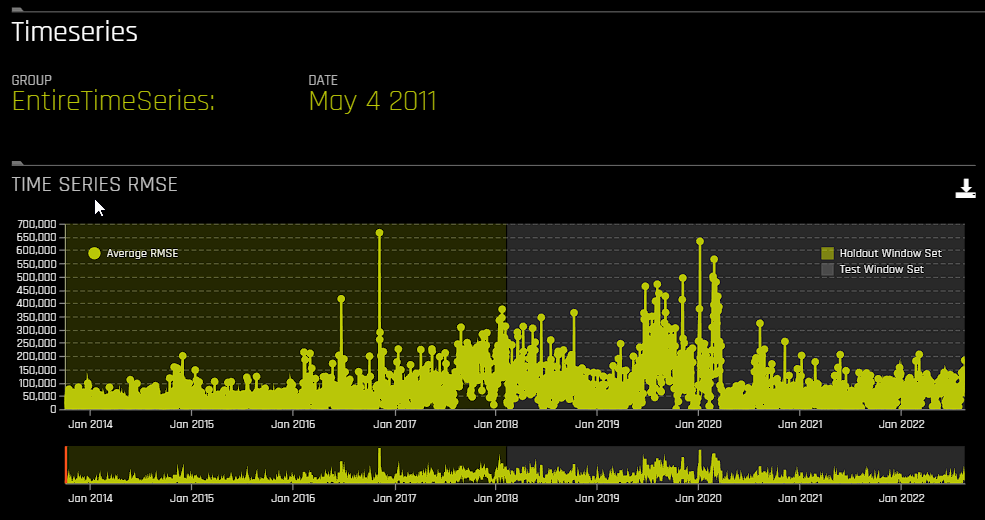• Actual vs. Predicted: View a graph that contrasts actual and predicted values. Note that this graph also features an accompanying slider that you can use to view a specific range of dates.

In addition to the preceding graphs, the following additional information is provided:

• Group metrics: Grouped metrics are based on an aggregation by group. For example, aggregate by store and department and get counts per group. You can also get the metric of interest, for example aggregate RMSE, etc. You can download all or specific group metrics by clicking the download button.

• Shapley values: Based on the selected date, Shapley values for each feature are provided in this section. To view Value + Bias for each feature and definitions of the transformed feature, click the Details button. You can also download Shapley values for the selected date by clicking the download button.

Note that you can select a specific group and / or date by clicking Group or Date.

### Sensitivity Analysis (SA)¶

#### Overview¶

Note: Sensitivity Analysis (SA) is only available for binary classification and regression experiments.

Sensitivity Analysis (or “What if?”) is a simple and powerful model debugging, explanation, fairness, and security tool. The idea behind SA is both direct and simple: Score your trained model on a single row, on multiple rows, or on an entire dataset of potentially interesting simulated values and compare the model’s new outcome to the predicted outcome on the original data.

Beyond traditional assessment practices, sensitivity analysis of machine learning model predictions is perhaps the most important validation technique for machine learning models. Sensitivity analysis investigates whether model behavior and outputs remain stable when data is intentionally perturbed or other changes are simulated in the data. Machine learning models can make drastically differing predictions for only minor changes in input variable values. For example, when looking at predictions that determine financial decisions, SA can be used to help you understand the impact of changing the most important input variables and the impact of changing socially sensitive variables (such as Sex, Age, Race, etc.) in the model. If the model changes in reasonable and expected ways when important variable values are changed, this can enhance trust in the model. Similarly, if the model changes to sensitive variables have minimal impact on the model, then this is an indication of fairness in the model predictions.

This page utilizes the What If Tool for displaying the SA information.

• A summary of the experiment

• Predictions for a specified column. Change the column on the Y axis to view predictions for that column.

• The current working score set. This updates each time you rescore.

• A filter tool for filtering the analysis. Choose a different column, predictions, or residuals. Set the filter type (<, >, etc.). Choose to filter by False Positive, False Negative, True Positive, or True Negative.

• Scoring chart. Click the Rescore button after applying a filter to update the scoring chart. This chart also lets you add or remove variables, toggle the main chart aggregation, reset the data, and delete the global history while resetting the data.

• The current history of actions taken on this page. You can delete individual actions by selecting the action and then clicking the Delete button that appears.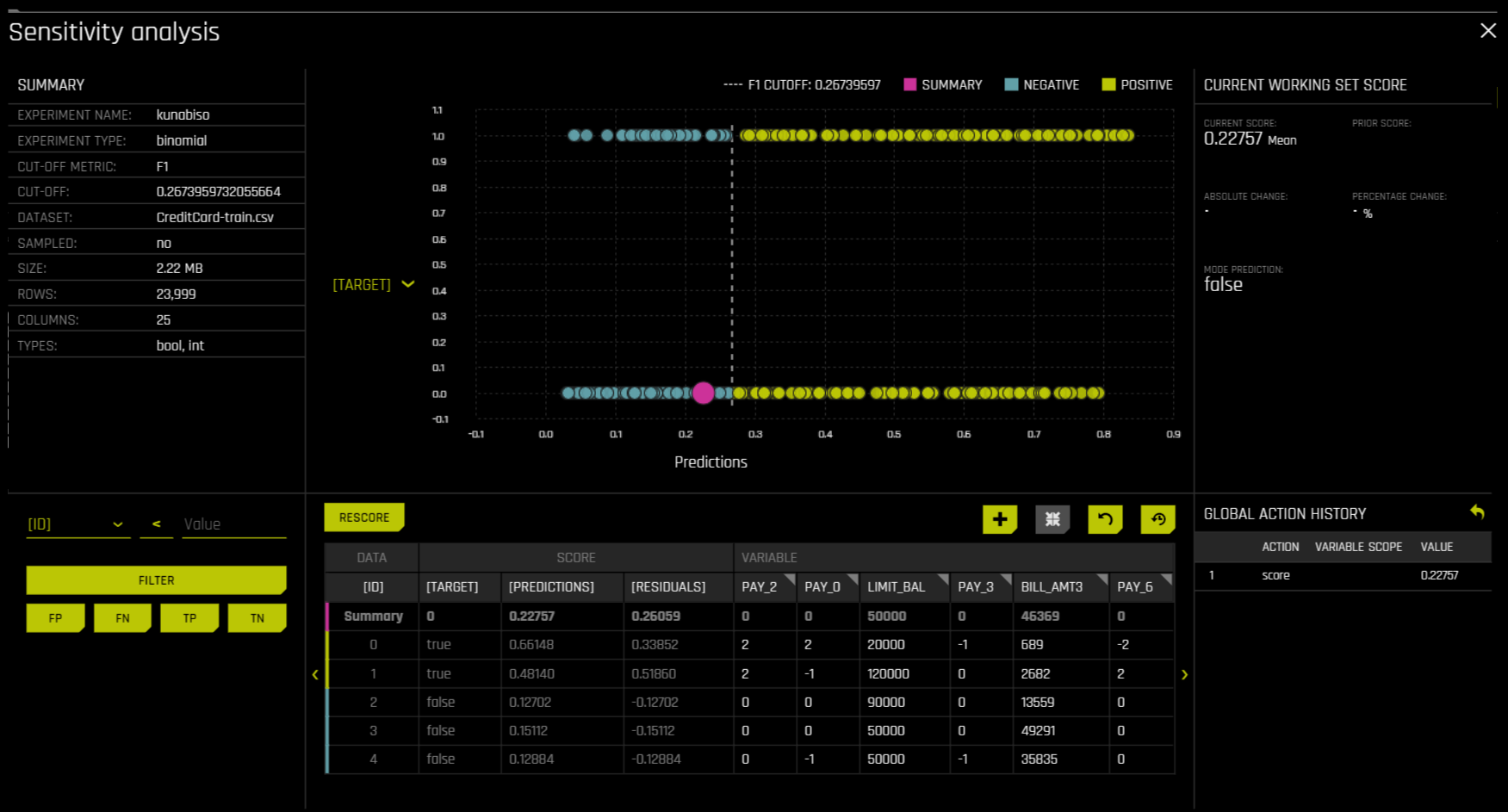#### Column actions¶

When clicking a column in SA, the following actions are available:

• Absolute: Change a column to a specific value for all rows. For example, you can set a column to have the value 5 for all observations. This is also possible for categorical columns. For example, you can set a categorical column to have the value “foobar” for all observations.

• Relative: Change a numeric column by adding a specific value to all rows. For example, you can add 9 to all observations in a numerical column. You can also pass in a negative number, for example, -9. The input must be numeric.

• Percentage: Change a numeric column by some percentage. For example, passing 9 to this field changes all values to be 9% of its original value. For example, if the value is 2 and you pass in 9 as the percentage, then the value changes to be 0.18. The input must be an integer.

• Set: Run the selected action with the valid value in the textbox.

• Randomize: Randomly change the values in a column, irrespective of what is in the textbox. The change itself is absolute and based on the domain of the column.

The following steps describe how to save and download a filtered dataset in SA.

1. Navigate to SA.

2. Edit a dataset, and then click the save icon to save the dataset.

3. After saving the filtered dataset, it is made available on the Datasets page.

#### Understand residuals¶

Residuals are differences between observed and predicted values. In Sensitivity Analysis, the method used to calculate residuals varies depending on the type of problem. For classification problems, logloss residuals are calculated for the class of interest. For regression problems, residuals are determined by calculating the square of the difference between targeted and predicted values.

#### Use cases¶

Use Case 1: Using SA on a Single Row or on a Small Group of Rows

This section describes scenarios for using SA for explanation, debugging, security, or fairness when scoring a trained model on a single row or on a small group of rows.

• Explanation: Change values for a variable, and then rescore the model. View the difference between the original prediction and the new model prediction. If the change is big, then the changed variable is locally important.

• Debugging: Change values for a variable, and then rescore the model. View the difference between the original prediction and the new model prediction and determine whether the change to variable made the model more or less accurate.

• Security: Change values for a variable, and then rescore the model. View the difference between the original prediction and the new model prediction. If the change is big, then the user can, for example, inform their IT department that this variable can be used in an adversarial attack or inform the model makers that this variable should be more regularized.

• Fairness: Change values for a demographic variable, and then rescore the model. View the difference between the original prediction and the new model prediction. If change is big, then the user can consider using a different model, regularizing the model more, or applying post-hoc bias remediation techniques.

• Random: Set variables to random values, and then rescore the model. This can help you look for things the you might not have thought of.

Use Case 2: Using SA on an Entire Dataset and Trained Model

This section describes scenarios for using SA for explanation, debugging, security, or fairness when scoring a trained model for an entire dataset and trained predictive model.

• Financial Stress Testing: Assume the user wants to see how their loan default rates will change (according to their trained probability of default model) when they change an entire dataset to simulate that all their customers are under more financial stress (such as lower FICO scores, lower savings balances, higher unemployment, etc). Change the values of the variables in their entire dataset, and look at the Percentage Change in the average model score (default probability) on the original and new data. They can then use this discovered information along with external information and processes to understand whether their institution has enough cash on hand to be prepared for the simulated crisis.

• Random: Set variables to random values, and then rescore the model. This lets users look for things they may not have otherwise considered.

Sensitivity Analysis on a Driverless AI Model: This ipynb uses the UCI credit card default data to perform sensitivity analysis and test model performance.

### Permutation Feature Importance¶

Note

• This plot is only available for binary classification and regression experiments.

• When permutation importance is enabled for interpretations, it is run as part of the interpretation process, regardless of whether it was run for the original experiment or AutoDoc.

Permutation-based feature importance shows how much a model’s performance would change if a feature’s values were permuted. If the feature has little predictive power, shuffling its values should have little impact on the model’s performance. If a feature is highly predictive, however, shuffling its values should decrease the model’s performance. The difference between the model’s performance before and after permuting the feature provides the feature’s absolute permutation importance.

## Surrogate Model Plots¶

This section describes the plots that are available in the Surrogate Model Tab.

### K-LIME and LIME-SUP¶

The MLI screen includes a K-LIME (K local interpretable model-agnostic explanations) or LIME-SUP (Locally Interpretable Models and Effects based on Supervised Partitioning) graph. A K-LIME graph is available by default when you interpret a model from the experiment page. When you create a new interpretation, you can instead choose to use LIME-SUP as the LIME method. Note that these graphs are essentially the same, but the K-LIME/LIME-SUP distinction provides insight into the LIME method that was used during model interpretation.

#### The K-LIME Technique¶

This plot is available for binary classification and regression models.

Summary

K-LIME creates one global surrogate GLM on the entire training data and also creates numerous local surrogate GLMs on samples formed from k-means clusters in the training data. The parameters of the global K-LIME model give an indication of overall linear feature importance and the overall average direction in which an input variable influences the Driverless AI model predictions. The in-cluster linear model parameters can be used to profile the local region, to give an average description of the important variables in the local region, and to understand the average direction in which an input variable affects the Driverless AI model predictions.

K-LIME is a variant of the LIME technique proposed by Ribeiro at al (2016). K-LIME generates global and local explanations that increase the transparency of the Driverless AI model, and allow model behavior to be validated and debugged by analyzing the provided plots, and comparing global and local explanations to one-another, to known standards, to domain knowledge, and to reasonable expectations.

As described in the preceding section, K-LIME creates numerous local surrogate GLMs on samples formed from k-means clusters in the training data. The features used for k-means are selected from the Random Forest surrogate model’s variable importance. The number of features used for k-means is the minimum of the top 25% of variables from the Random Forest surrogate model’s variable importance and the max number of variables that can be used for k-means, which is set by the user in the config.toml setting for mli_max_number_cluster_vars. (Note that if the number of features in the dataset are less than or equal to 6, then all features are used for k-means clustering.) The previous setting can be turned off to use all features for k-means by setting use_all_columns_klime_kmeans in the config.toml file to true. All penalized GLM surrogates are trained to model the predictions of the Driverless AI model.

The number of clusters for local explanations is chosen by a grid search in which the $$R^2$$ between the Driverless AI model predictions and all of the local K-LIME model predictions is maximized. The global and local linear model’s intercepts, coefficients, $$R^2$$ values, accuracy, and predictions can all be used to debug and develop explanations for the Driverless AI model’s behavior. In addition to the usage described in the preceding section, the global model is also used to generate explanations for very small clusters ($$N < 20$$) where fitting a local linear model is inappropriate.

As described in the preceding section, the in-cluster linear model parameters can be used to profile the local region, to give an average description of the important variables in the local region, and to understand the average direction in which an input variable affects the Driverless AI model predictions. For a point within a cluster, the sum of the local linear model intercept and the products of each coefficient with their respective input variable value are the K-LIME prediction. By disaggregating the K-LIME predictions into individual coefficient and input variable value products, the local linear impact of the variable can be determined. This product is sometimes referred to as a reason code and is used to create explanations for the Driverless AI model’s behavior.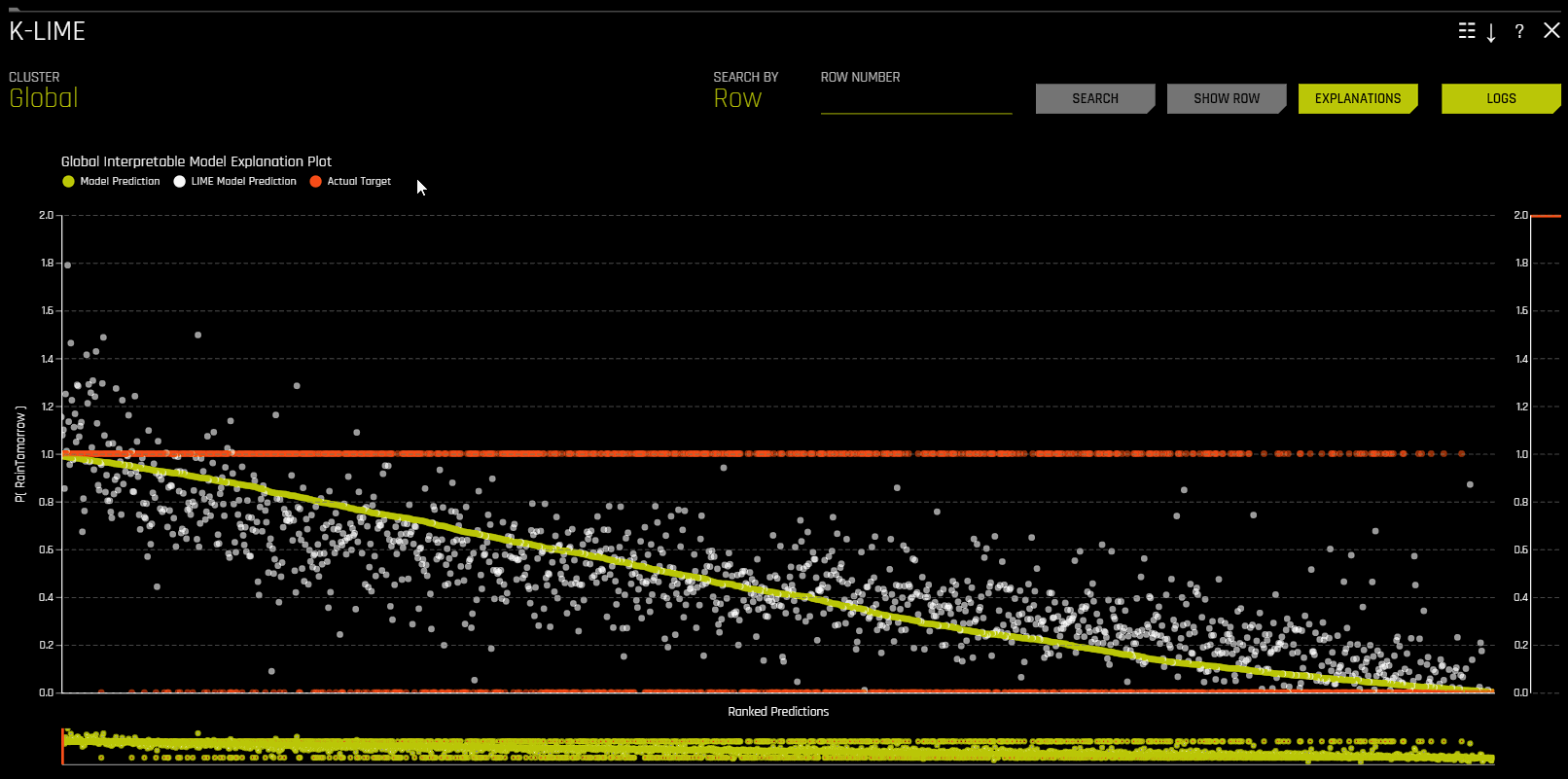Reason codes in K-LIME

The K-LIME plot includes a Reason codes page that can be accessed by clicking the Explanations button. From the Reason codes page, you can view information about both cluster-specific reason codes and global reason codes.

In K-LIME, reason code values are calculated by determining each coefficient-feature product. Reason code values are also written into automatically generated reason codes, available in the local reason code section of the explanations dialog.

In the following example, reason codes are created by evaluating and disaggregating a local linear model.

Given the row of input data with its corresponding Driverless AI and K-LIME predictions:

debt_to_income_ ratio

credit_ score

savings_acct_ balance

observed_ default

H2OAI_predicted_ default

K-LIME_predicted_ default

30

600

1000

1

0.85

0.9

And the local linear model:

$$\small{y_\text{K-LIME} = 0.1 + 0.01 * debt\_to\_income\_ratio + 0.0005 * credit\_score + 0.0002 * savings\_account\_balance}$$

It can be seen that the local linear contributions for each variable are:

• debt_to_income_ratio: 0.01 * 30 = 0.3

• credit_score: 0.0005 * 600 = 0.3

• savings_acct_balance: 0.0002 * 1000 = 0.2

Each local contribution is positive and thus contributes positively to the Driverless AI model’s prediction of 0.85 for H2OAI_predicted_default. By taking into consideration the value of each contribution, reason codes for the Driverless AI decision can be derived. debt_to_income_ratio and credit_score would be the two largest negative reason codes, followed by savings_acct_balance.

The local linear model intercept and the products of each coefficient and corresponding value sum to the K-LIME prediction. Moreover it can be seen that these linear explanations are reasonably representative of the nonlinear model’s behavior for this individual because the K-LIME predictions are within 5.5% of the Driverless AI model prediction. This information is encoded into English language rules which can be viewed by clicking the Explanations button.

Like all LIME explanations based on linear models, the local explanations are linear in nature and are offsets from the baseline prediction, or intercept, which represents the average of the penalized linear model residuals. Of course, linear approximations to complex non-linear response functions will not always create suitable explanations and users are urged to check the K-LIME plot, the local model $$R^2$$, and the accuracy of the K-LIME prediction to understand the validity of the K-LIME local explanations. When K-LIME accuracy for a given point or set of points is quite low, this can be an indication of extremely nonlinear behavior or the presence of strong or high-degree interactions in this local region of the Driverless AI response function. In cases where K-LIME linear models are not fitting the Driverless AI model well, nonlinear LOCO feature importance values may be a better explanatory tool for local model behavior. As K-LIME local explanations rely on the creation of k-means clusters, extremely wide input data or strong correlation between input variables may also degrade the quality of K-LIME local explanations.

#### The LIME-SUP Technique¶

This plot is available for binary classification and regression models.

LIME-SUP explains local regions of the trained Driverless AI model in terms of the original variables. Local regions are defined by each leaf node path of the decision tree surrogate model instead of simulated, perturbed observation samples - as in the original LIME. For each local region, a local GLM model is trained on the original inputs and the predictions of the Driverless AI model. Then the parameters of this local GLM can be used to generate approximate, local explanations of the Driverless AI model.

The Global Interpretable Model Explanation Plot

This plot shows Driverless AI model predictions and LIME model predictions in sorted order by the Driverless AI model predictions. This graph is interactive. Hover over the Model Prediction, LIME Model Prediction, or Actual Target radio buttons to magnify the selected predictions. Or click those radio buttons to disable the view in the graph. You can also hover over any point in the graph to view LIME reason codes for that value. By default, this plot shows information for the global LIME model, but you can change the plot view to show local results from a specific cluster. The LIME plot also provides a visual indication of the linearity of the Driverless AI model and the trustworthiness of the LIME explanations. The closer the local linear model approximates the Driverless AI model predictions, the more linear the Driverless AI model and the more accurate the explanation generated by the LIME local linear models.

### Surrogate Decision Tree¶

The decision tree surrogate model increases the transparency of the Driverless AI model by displaying an approximate flow-chart of the complex Driverless AI model’s decision making process. It also displays the most important variables in the Driverless AI model and the most important interactions in the Driverless AI model. The decision tree surrogate model can be used for visualizing, validating, and debugging the Driverless AI model by comparing the displayed decision-process, important variables, and important interactions to known standards, domain knowledge, and reasonable expectations. It is known to date back at least to 1996 (Craven and Shavlik).

A surrogate model is a data mining and engineering technique in which a generally simpler model is used to explain another usually more complex model or phenomenon. Given our learned function $$g$$ and set of predictions, $$g(X) = \hat{Y}$$, we can train a surrogate model $$h$$: $$X,\hat{Y} \xrightarrow{\mathcal{A}_{\text{surrogate}}} h$$, such that $$h(X)$$ is approximately equal to $$g(X)$$. To preserve interpretability, the hypothesis set for $$h$$ is often restricted to linear models or decision trees.

For the purposes of interpretation in Driverless AI, $$g$$ is considered to represent the entire pipeline, including both the feature transformations and model, and the surrogate model is a decision tree ($$h_{\text{tree}}$$). Users must also note that there exist few guarantees that $$h_{\text{tree}}$$ accurately represents $$g$$. The RMSE for $$h_{\text{tree}}$$ is displayed for assessing the fit between $$h_{\text{tree}}$$ and $$g$$.

$$h_{\text{tree}}$$ is used to increase the transparency of $$g$$ by displaying an approximate flow chart of the decision making process of $$g$$ as displayed in the following image:

$$h_{\text{tree}}$$ also shows the likely important features and the most important interactions in $$g$$. $$h_{\text{tree}}$$ can be used for visualizing, validating, and debugging $$g$$ by comparing the displayed decision-process, important features, and important interactions to known standards, domain knowledge, and reasonable expectations.

The preceding image displays the decision tree surrogate, $$h_{\text{tree}}$$, for an example probability of default model, $$g$$, created with Driverless AI using the UCI repository credit card default data (see https://www.kaggle.com/uciml/default-of-credit-card-clients-dataset). The PAY_0 feature is likely the most important feature in $$g$$ due to its place in the initial split in $$h_{\text{tree}}$$ and its second occurrence on the third level of $$h_{\text{tree}}$$. First level interactions between PAY_0 and PAY_2 and between PAY_0 and PAY_5 are visible along with several second level interactions. Following the decision path to the lowest probability leaf node in $$h_{\text{tree}}$$ (lower left in the preceding image) shows that customers who pay their first (PAY_0) and second (PAY_2) month bills on time are the least likely to default according to $$h_{\text{tree}}$$. The thickness of the edges in this path indicate that this is a very common decision path through $$h_{\text{tree}}$$. Following the decision path to the highest probability leaf node in $$h_{\text{tree}}$$ (second from right in the preceding image) shows that customers who are late on their first (PAY_0) and fifth (PAY_5) month bills and who pay less than 16520 in their sixth payment (PAY_AMT6) are the most likely to default according to $$h_{\text{tree}}$$. The thinness of the edges in this path indicate that this is a relatively rare decision path through $$h_{\text{tree}}$$. When an observation of data is selected using the k-LIME plot, $$h_{\text{tree}}$$ can also provide a degree of local interpretability. When a single observation, $$x^{(i)}$$, is selected, its path through $$h_{\text{tree}}$$ is highlighted. The path of $$x^{(i)}$$ through $$h_{\text{tree}}$$ can be helpful when analyzing the logic or validity of $$g(x^{(i)})$$.

#### MLI Taxonomy: Decision Tree Surrogate Models¶

• Scope of Interpretability:

1. Generally, decision tree surrogates provide global interpretability.

1. The attributes of a decision tree are used to explain global attributes of a complex Driverless AI model such as important features, interactions, and decision processes.

• Appropriate Response Function Complexity: Decision tree surrogate models can create explanations for models of nearly any complexity.

• Understanding and Trust:

1. Decision tree surrogate models foster understanding and transparency because they provide insight into the internal mechanisms of complex models.

1. They enhance trust, accountability, and fairness when their important features, interactions, and decision paths are in line with human domain knowledge and reasonable expectations.

• Application Domain: Decision tree surrogate models are model agnostic.

#### Surrogate Decision Tree Plot¶

This plot is available for binary and multiclass classification models as well as regression models.

In the Decision Tree plot, the highlighted row shows the path to the highest probability leaf node and indicates the globally important variables and interactions that influence the Driverless AI model prediction for that row. You can view rules for a specific path by clicking the path’s terminal node.

Note: For a list of Surrogate Decision Tree explainer expert settings, see Surrogate Decision Tree Explainer Settings.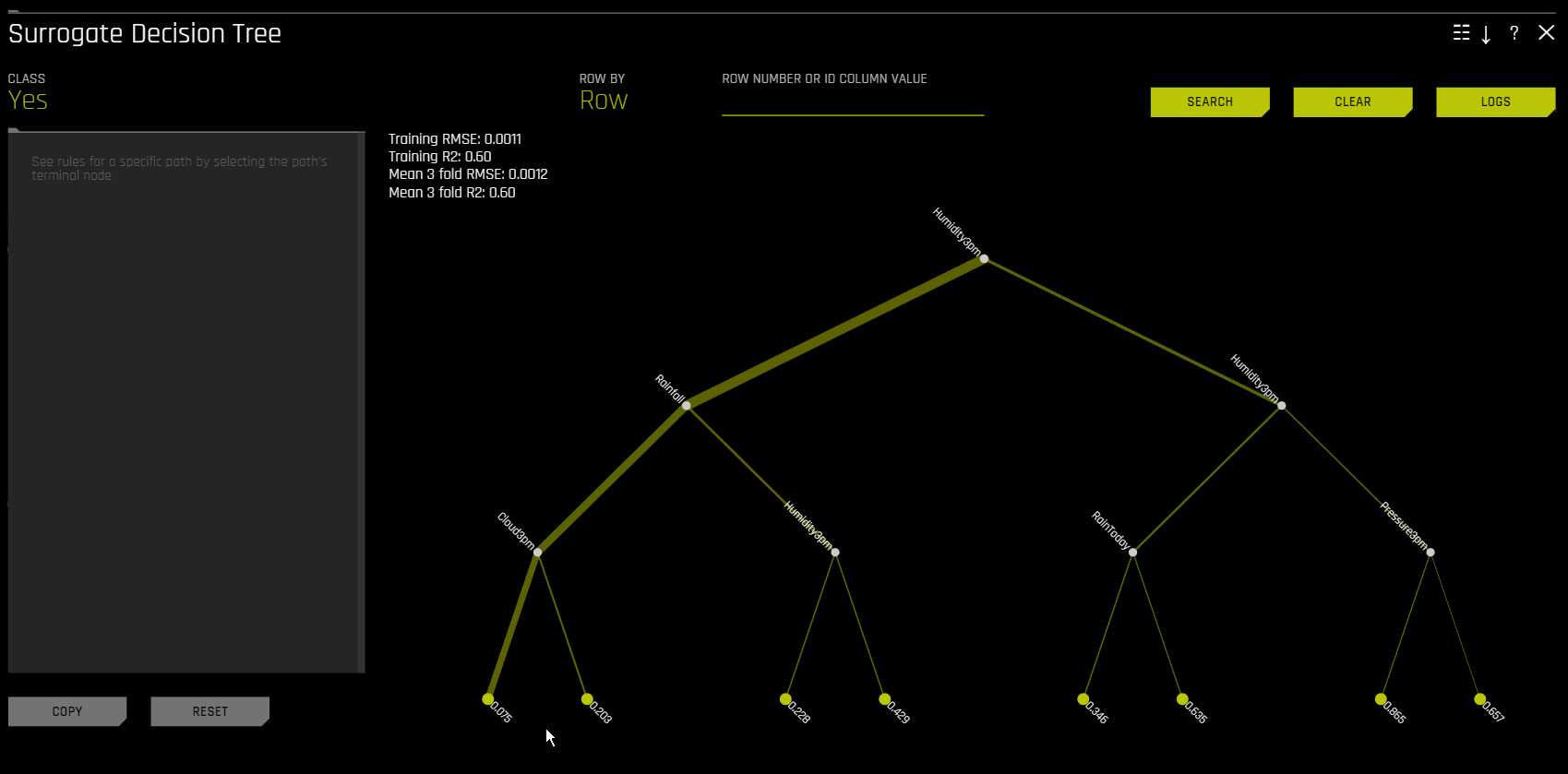For multiclass models, decision trees are created for each class. To view a decision tree for a specific class, click Class in the upper-left corner of the page and select the class you want to view a decision tree for.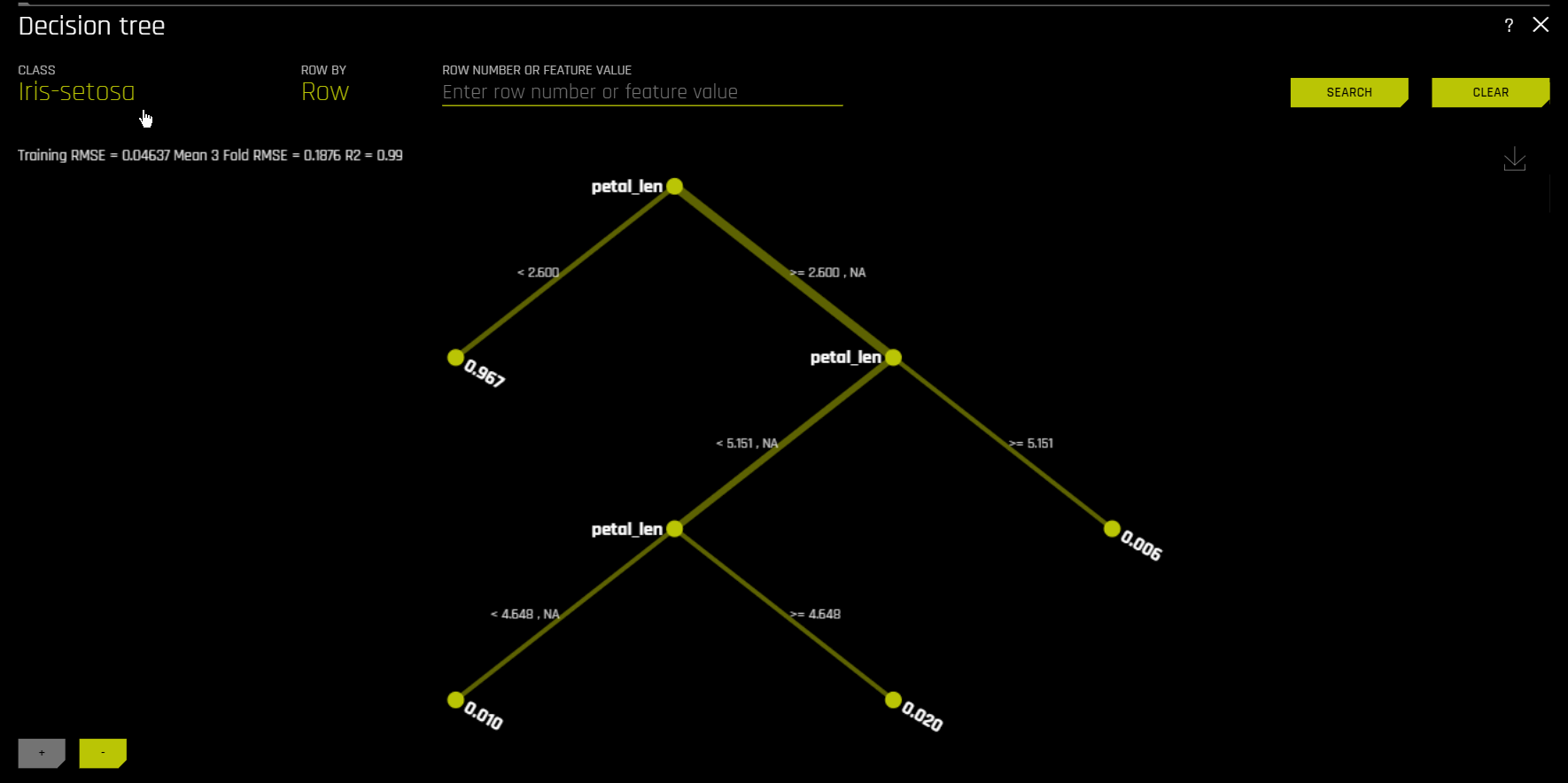You can view a predictive performance chart for the Decision Tree surrogate model by clicking the Performance button. This chart lets you evaluate the performance of the surrogate DT model. The white dots in the performance chart represent the surrogate model predictions. To return to the Decision Tree view, click the Tree button. Note that this feature is not supported for multinomial experiments.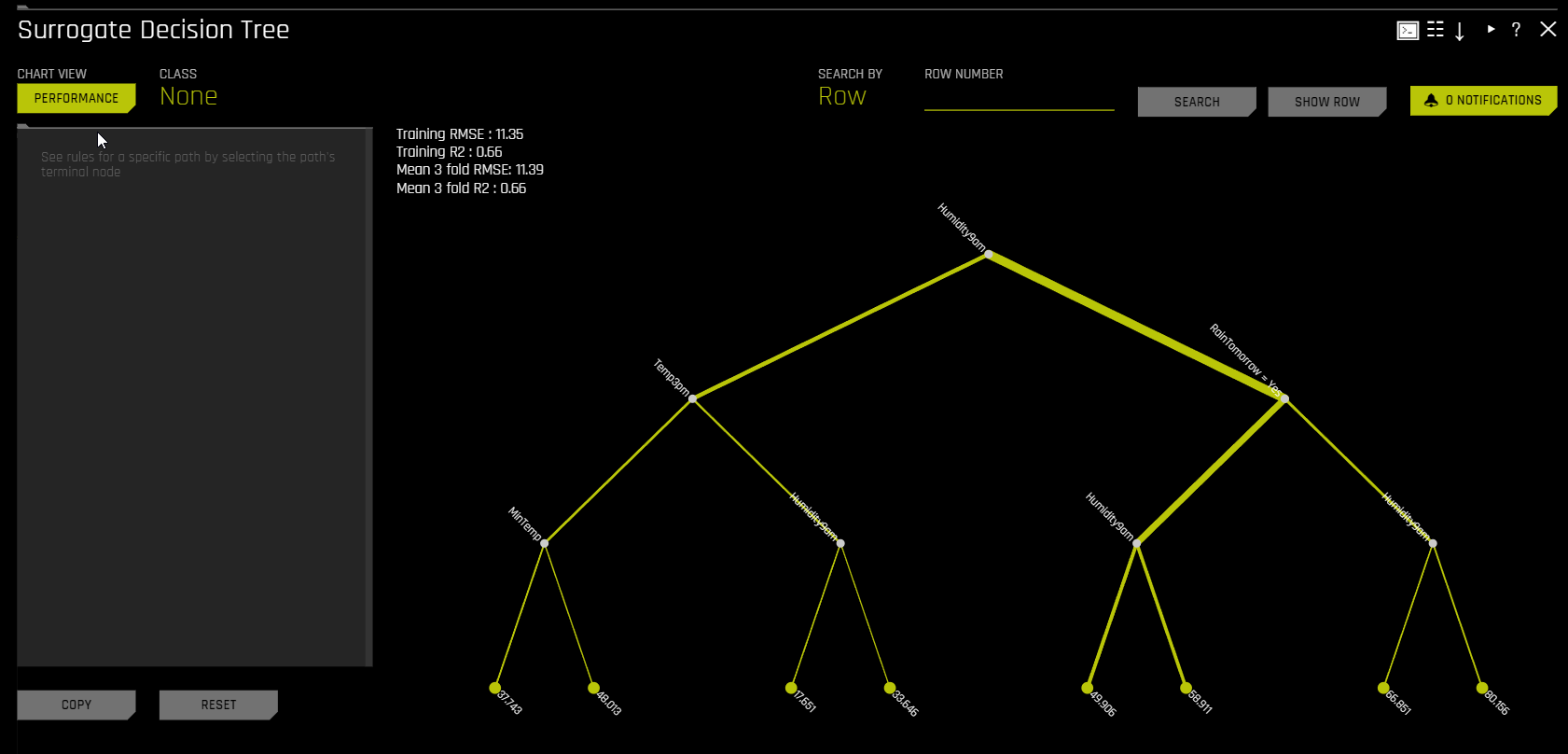### Random Forest surrogate model plots¶

The following sections describe the available Random Forest surrogate model plots.

Note: You can view a predictive performance chart for each of the Random Forest surrogate models by clicking the Performance button after clicking on the explainer tile. This chart lets you evaluate the performance of that specific Random Forest surrogate model. The white dots in the performance chart represent the surrogate model predictions. To return to the main view, click the Back to Main button. Note that this feature is not supported for multinomial experiments.

#### Random Forest Feature Importance¶

This plot is available for all models for binary classification, multiclass classification, and regression experiments.

Global Feature Importance vs Local Feature Importance

Global feature importance (yellow) is a measure of the contribution of an input variable to the overall predictions of the Driverless AI model. Global feature importance is calculated by aggregating the improvement in splitting criterion caused by a single variable across all of the decision trees in the Random Forest surrogate model.

Local feature importance (grey) is a measure of the contribution of an input variable to a single prediction of the Driverless AI model. Local feature importance values for regression and binomial cases are calculated by tracing single rows of data through the random forest surrogate model and returning the absolute LOCO values. For the multiclass case, local feature importance values are calculated by re-scoring the trained supervised model and measuring the impact of setting each variable to missing. The absolute value of differences across classes is then calculated for each dropped or replaced column.

Both global and local variable importance are scaled so that the largest contributor has a value of 1.

Note: Engineered features are used for MLI when a time series experiment is built. This is because munged time series features are more useful features for MLI than raw time series features, as raw time series features are not IID (Independent and Identically Distributed).

#### Random Forest Partial Dependence and Individual Conditional Expectation¶

A Partial Dependence and ICE plot is available for both Driverless AI and surrogate models. Refer to the previous Partial Dependence (PDP) and Individual Conditional Expectation (ICE) section for more information about this plot.

#### Random Forest LOCO¶

This plot is available for binary and multiclass classification models as well as regression models.

Local feature importance describes how the combination of the learned model rules or parameters and an individual row’s attributes affect a model’s prediction for that row while taking nonlinearity and interactions into effect. Local feature importance values reported in this plot are based on a variant of the leave-one-covariate-out (LOCO) method (Lei et al, 2017).

The LOCO-variant method for binary and regression models is calculated by traversing the random forest surrogate model and removing the prediction contribution of any rule containing the variable of interest for every tree from the original prediction. Local LOCO values are calculated by tracing single rows of data through the random forest surrogate model. Global LOCO values are the average of the LOCO values over every row of a dataset.

The LOCO-variant method for multiclass models differs slightly in that it calculates row-wise local feature importance values by re-scoring the trained supervised model and measuring the impact of setting each variable to missing. The sum of the absolute value of differences across classes is then calculated for each dropped or replaced column.

Given the row of input data with its corresponding Driverless AI and K-LIME predictions:

debt_to_income_ ratio

credit_ score

savings_acct_ balance

observed_ default

H2OAI_predicted_ default

K-LIME_predicted_ default

30

600

1000

1

0.85

0.9

Taking the Driverless AI model as F(X), LOCO-variant feature importance values are calculated as follows.

First, the modified predictions are calculated:

$$F_{~debt\_to\_income\_ratio} = F(NA, 600, 1000) = 0.99$$

$$F_{~credit\_score} = F(30, NA, 1000) = 0.73$$

$$F_{~savings\_acct\_balance} = F(30, 600, NA) = 0.82$$

Second, the original prediction is subtracted from each modified prediction to generate the unscaled local feature importance values:

$$\text{LOCO}_{debt\_to\_income\_ratio} = F_{~debt\_to\_income\_ratio} - 0.85 = 0.99 - 0.85 = 0.14$$

$$\text{LOCO}_{credit\_score} = F_{~credit\_score} - 0.85 = 0.73 - 0.85 = -0.12$$

$$\text{LOCO}_{savings\_acct\_balance} = F_{~savings\_acct\_balance} - 0.85 = 0.82 - 0.85 = -0.03$$

Finally LOCO values are scaled between 0 and 1 by dividing each value for the row by the maximum value for the row and taking the absolute magnitude of this quotient.

$$\text{Scaled}(\text{LOCO}_{debt\_to\_income\_ratio}) = \text{Abs}(\text{LOCO}_{~debt\_to\_income\_ratio}/0.14) = 1$$

$$\text{Scaled}(\text{LOCO}_{credit\_score}) = \text{Abs}(\text{LOCO}_{~credit\_score}/0.14) = 0.86$$

$$\text{Scaled}(\text{LOCO}_{savings\_acct\_balance}) = \text{Abs}(\text{LOCO}_{~savings\_acct\_balance} / 0.14) = 0.21$$

One drawback to these LOCO-variant feature importance values is, unlike K-LIME, it is difficult to generate a mathematical error rate to indicate when LOCO values may be questionable.

### NLP Surrogate Models¶

These plots are available for natural language processing (NLP) models.

For NLP surrogate models, Driverless AI creates a TF-IDF matrix by tokenizing all text features. The resulting frame is appended to numerical or categorical columns from the training dataset, and the original text columns are removed. This frame is then used for training surrogate models that have prediction columns consisting of tokens and the original numerical or categorical features.

Notes:

• MLI support for NLP is only available for binary classification and regression experiments.

• Each row in the TF-IDF matrix contains $$N$$ columns, where $$N$$ is the total number of tokens in the corpus with values that are appropriate for that row (0 if absent).

• Driverless AI does not currently generate a K-LIME scoring pipeline for MLI NLP problems.

#### Running Surrogate Models on Residuals¶

In Driverless AI, residuals (that is, the differences between the actual observed values and the predicted values of a machine learning model) can be used as targets in MLI surrogate models for the purpose of debugging models. The method used to calculate residuals varies depending on the type of problem. For classification problems, logloss residuals are calculated for a specified class. For regression problems, residuals are determined by calculating the square of the difference between targeted and predicted values.

The following steps describe how you can run MLI surrogate models on residuals:

1. In the main navigation menu, click Experiments and select a completed experiment.

2. On the completed experiment page, click Interpret this model > With custom settings.

3. On the Interpretation Settings page, click Expert Settings.

4. In the Interpretation Expert Settings panel, click the MLI Model Debugging tab. Enable the Debug model residuals with surrogate models interpretation expert setting.

5. For classification experiments, specify a class to use as an outcome of interest with the Class for debugging classification model logloss residuals interpretation expert setting. (Note that this setting is not visible for regression problems. For classification problems, this setting is only displayed once the Debug model residuals with surrogate models interpretation expert setting is enabled.)

6. Click Save to confirm your selection, and then click Launch MLI. Note that the predicted values that were generated by the selected model are used when calculating residuals.

7. Once the interpretation is complete, you can view the models from the explanations page by clicking the Surrogate Models on Residuals tab.

## NLP Plots¶

This section describes the plots that are available in the NLP tab.

Note

• The following plots are only available for natural language processing (NLP) models.

### NLP Leave-One-Covariate-Out (LOCO)¶

This plot is available for binomial, multiclass, and regression natural language processing (NLP) models. It is located in the NLP tab on the Model Interpretation page, which is only visible for NLP models.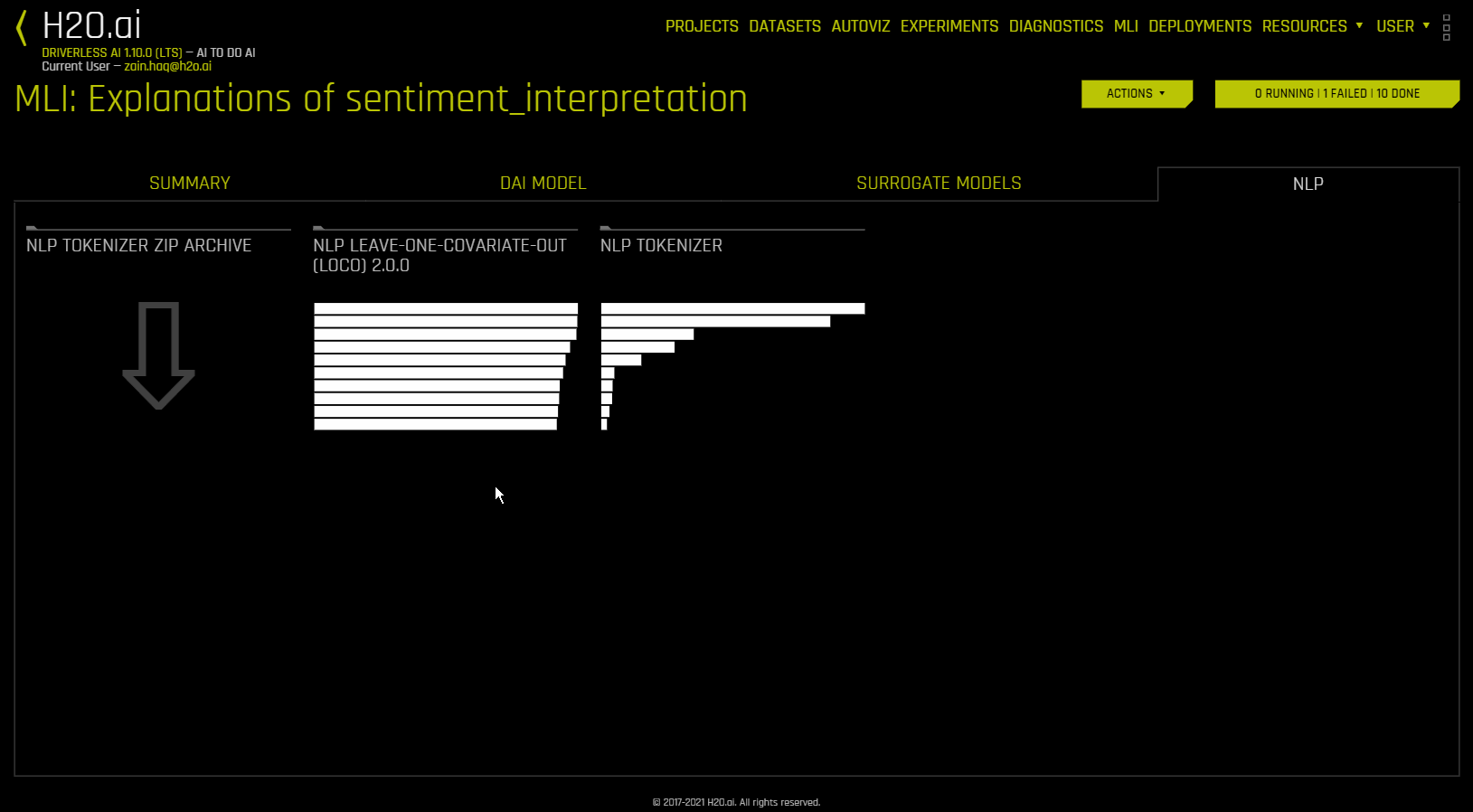This plot applies a leave-one-covariate-out (LOCO) styled approach to NLP models by removing a specific token, which is obtained by TF-IDF, from only a single column where the token is occurring. For example, if there is a token foo in both column1 and column2, LOCO is computed for both columns separately, even though the token is the same. Note that the TF-IDF for the token differs in both columns. In addition, if a token does not exist in a row, then it is appended before calculating LOCO to ensure the token was evaluated across all rows. The difference between the resulting score and the original score (token included) is useful when trying to determine how specific changes to text features alter the predictions made by the model. Driverless AI fits a separate TF-IDF vectorizer for each individual column and concatenates the results. The terms (tokens) in the resulting importance frames are then wrapped with column names:

Column Names Example

column1(‘and’)

column1(‘apple’)

column2(‘and’)

0.1

0.0005

0.412512

The NLP LOCO plot lets you view text for a specific row by specifying a row number. Each token in the row is highlighted by importance. You can switch between different text features and view their respective importances globally and locally.

Note

• Due to computational complexity, the global importance value is only calculated for $$N$$ (20 by default) tokens. This value can be changed with the mli_nlp_top_n configuration option.

• A specific token selection method can be used by specifying one of the following options for the mli_nlp_min_token_mode configuration option:

• linspace: Selects $$N$$ evenly spaced tokens according to their TF-IDF score (Default)

• top: Selects top $$N$$ tokens by TF-IDF score

• bottom: Selects bottom $$N$$ tokens by TF-IDF score

• Local values for NLP LOCO can take a significant amount of time to calculate depending on the specifications of your hardware.

• Driverless AI does not currently generate a K-LIME scoring pipeline for MLI NLP problems.

### NLP Partial Dependence Plot¶

This plot is available for binomial, multiclass, and regression natural language processing (NLP) models. It is located in the NLP tab on the Model Interpretation page, which is only visible for NLP models.

NLP partial dependence (yellow) portrays the average prediction behavior of the Driverless AI model when an input text token is left in its respective text and not included in its respective text along with +/- 1 standard deviation bands. ICE (grey) displays the prediction behavior for an individual row of data when an input text token is left in its respective text and not included in its respective text. The text tokens are generated from TF-IDF.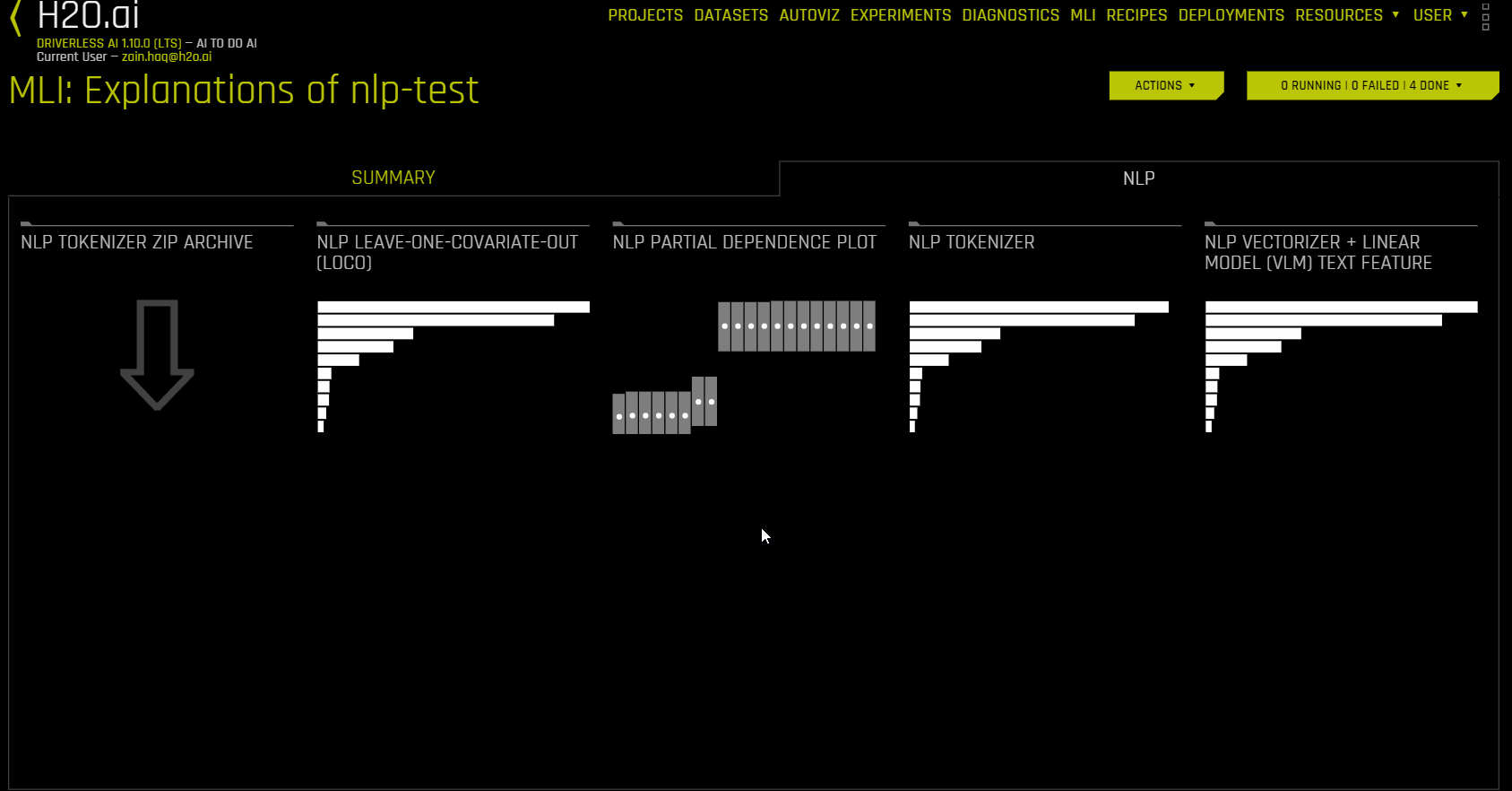### NLP Tokenizer¶

This plot is available for natural language processing (NLP) models. It is located in the NLP tab on the Model Interpretation page, which is only visible for NLP models.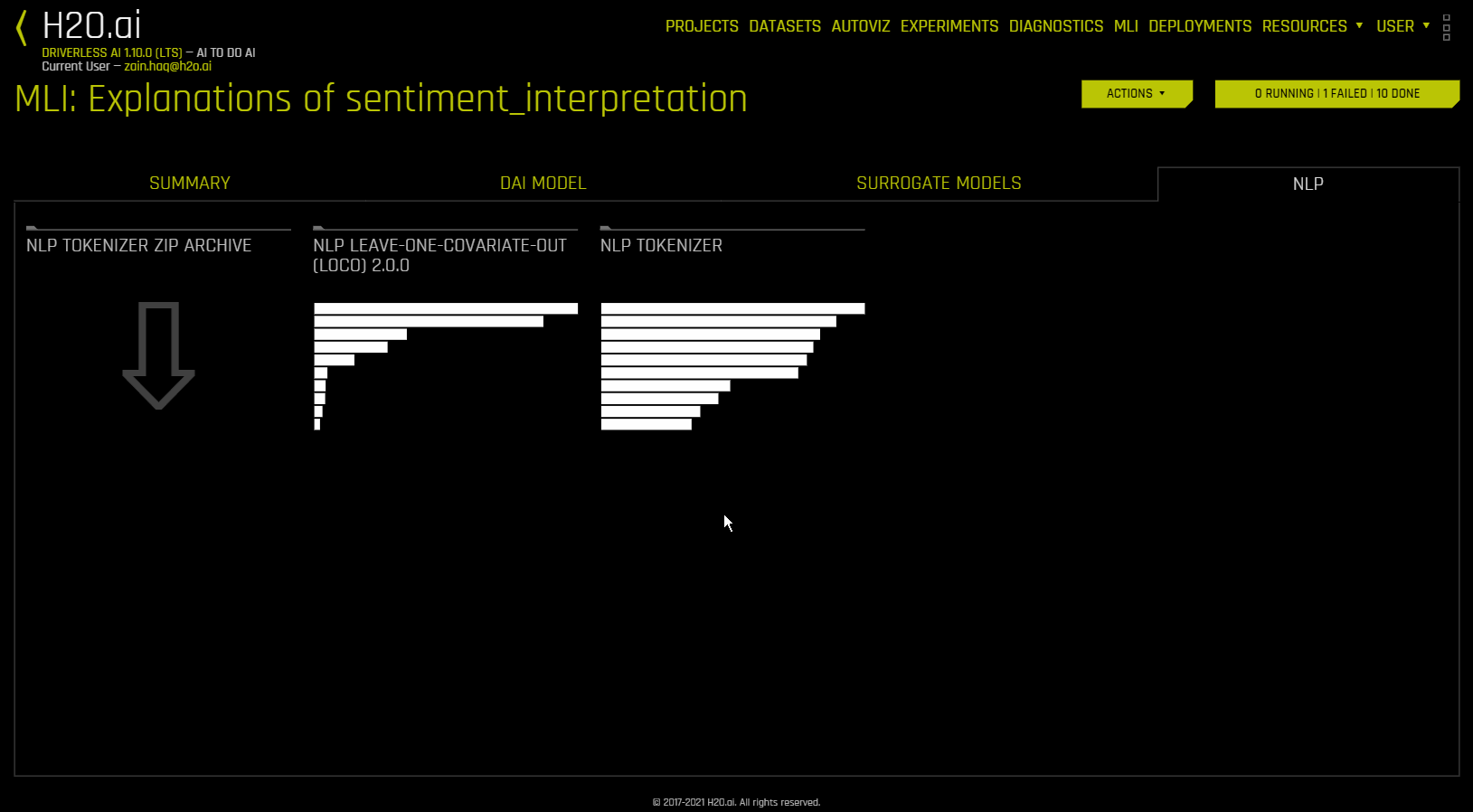This plot shows both the global and local importance values of each token in a corpus (a large and structured set of texts). The corpus is automatically generated from text features used by Driverless AI models prior to the process of tokenization.

Local importance values are calculated by using the term frequency–inverse document frequency (TF-IDF) as a weighting factor for each token in each row. The TF-IDF increases proportionally to the number of times a token appears in a given document and is offset by the number of documents in the corpus that contain the token. Specify the row that you want to view, then click the Search button to see the local importance of each token in that row.

Global importance values are calculated by using the inverse document frequency (IDF), which measures how common or rare a given token is across all documents. (Default View)

You can download an archive of files relating to the NLP Tokenizer plot by clicking “NLP Tokenizer ZIP Archive” in the NLP tab.

Note

• MLI for NLP does not currently feature the option to remove stop words.

• By default, up to 10,000 tokens are created during the tokenization process. This value can be changed in the configuration.

• By default, Driverless AI uses up to 10,000 documents to extract tokens from. This value can be changed with the config.mli_nlp_sample_limit parameter. Downsampling is used for datasets that are larger than the default sample limit.

• Driverless AI does not currently generate a K-LIME scoring pipeline for MLI NLP problems.

• With the LOCO method, a specific token is removed from only a single column where the token is occurring. For example, if there is a token foo in both column1 and column2, LOCO is computed for both columns separately, even though the token is the same. The TF-IDF for the token differs in both columns.

### NLP Vectorizer + Linear Model (VLM) Text Feature Importance¶

This plot is available for binomial and regression natural language processing (NLP) models. It is located in the NLP tab on the Model Interpretation page, which is only visible for NLP models.

NLP Vectorizer + Linear Model (VLM) text feature importance uses TF-IDF of individual words as features from a text column of interest and builds a linear model (currently GLM) using those features and fits it to either the predicted class (binary classification) or the continuous prediction (regression) of the Driverless AI model. The coefficients of the linear model give the importance of the words. Note that by default, this explainer uses the first text column based on alphabetical order.